# Learning from binary labels with instance-dependent noise

## Abstract

Supervised learning has seen numerous theoretical and practical advances over the last few decades. However, its basic assumption of identical train and test distributions often fails to hold in practice. One important example of this is when the training instances are subject to label noise: that is, where the observed labels do not accurately reflect the underlying ground truth. While the impact of simple noise models has been extensively studied, relatively less attention has been paid to the practically relevant setting of instance-dependent label noise. It is thus unclear whether one can learn, both in theory and in practice, good models from data subject to such noise, with no access to clean labels. We provide a theoretical analysis of this issue, with three contributions. First, we prove that for instance-dependent (but label-independent) noise, any algorithm that is consistent for classification on the noisy distribution is also consistent on the noise-free distribution. Second, we prove that consistency also holds for the area under the ROC curve, assuming the noise scales (in a precise sense) with the inherent difficulty of an instance. Third, we show that the Isotron algorithm can efficiently and provably learn from noisy samples when the noise-free distribution is a generalised linear model. We empirically confirm our theoretical findings, which we hope may stimulate further analysis of this important learning setting.

## Learning with instance-dependent label noise

Recent advances in classification models such as deep neural networks have seen resounding successes (Krizhevsky et al. 2012; He et al. 2016; Xiao et al 2015), in no small part due to the availability of large labelled training datasets. However, real-world labels are often corrupted by instance-dependent label noise, wherein the observed labels are not representative of the underlying ground truth, and noise levels vary across different instances. For example, in object recognition problems, poor quality images are more likely to be mislabelled (Reed et al. 2014; Xiao et al 2015); furthermore, certain classes of images tend to be confused with others. A natural question thus arises: what can we say about the impact of such label noise on the accuracy of our trained models?

More precisely, the following questions are of fundamental interest:

Q1 :

does good classification performance on the noisy distribution translate to good classification performance on the noise-free (“clean”) distribution?

Q2 :

does the answer to Q1 also hold for more complex measures, e.g. for ranking?

Q3 :

are there simple algorithms which are provably noise robust?

In the case of instance-independent label noise, questions Q1Q3 have been studied by several recent theoretical works (Stempfel et al. 2007; Stempfel and Ralaivola 2009; Natarajan et al. 2013; Scott et al. 2013; Liu and Tao 2015; Menon et al. 2015; van Rooyen et al. 2015; Patrini et al. 2016, 2017), whose analysis has resulted in a surprising conclusion: for powerful (high-capacity) models, one can achieve optimal classification and ranking error given enough noisy examples, without the need for any clean labels. Further, for modest (low-capacity) models, while even a tiny amount of noise may be harmful (Long and Servedio 2008), there are simple provably robust algorithms (Natarajan et al. 2013; van Rooyen et al. 2015).

In the case of instance-dependent label noise, while there is some theoretical precedent  (Manwani and Sastry 2013; Ghosh et al. 2015; Awasthi et al. 2015), questions Q1Q3 have to our knowledge remained unanswered. In this paper, we study these questions systematically. We answer Q1 and Q2 by showing that under (suitably constrained) instance-dependent noise, powerful models can optimally classify and rank given enough noisy samples; this is a non-trivial generalisation of existing results. We answer Q3 by showing how an existing algorithmic extension of generalised linear models can efficiently and provably learn from noisy samples; this is in contrast to existing algorithms even for instance-independent noise, which either require the noise rate to be known, or lack guarantees.

More precisely, our contributions are:

C1 :

we show that for a range of losses, any algorithm that minimises the expected loss (i.e., risk) on the noisy distribution also minimises the expected loss on the clean distribution (Theorem 1) i.e., noisy risk minimisation is consistent for classification;

C2 :

we show that area under the ROC curve (AUROC) maximisation on the noisy distribution is also consistent for the clean distribution (Theorem 2), under a new boundary-consistent noise model where “harder” instances are subject to noise (Definition 4);

C3 :

we show that if the clean distribution is a generalised linear model, the Isotron algorithm (Kalai and Sastry 2009) is provably robust to boundary-consistent noise (Theorem 3).

While our contributions are primarily of a theoretical nature, we also provide experiments (Sect. 7) illustrating potential practical implications of our results.

## Background and notation

We begin with some notation and background material. Table 1 provides a glossary.

### Learning from binary labels

In standard problems of learning from binary labels, one observes a set of instances paired with binary labels, assumed to be an i.i.d. draw from an unobserved distribution. The goal is to find a model that can determine if future instances are more likely to be positive or negative. To state this more formally, we need some notation.

#### Distributions, scorers, and risks

Fix a measurable instance space $$\mathscr {X}$$. We denote by $$D$$ some distribution over $$\mathscr {X}\times \{ \pm 1 \}$$, with random variables $$(\mathsf {X}, \mathsf {Y}) \sim D$$. Any $$D$$ may be expressed via the marginal $$M = \mathbb {P}( \mathsf {X})$$ and class-probability function $$\eta :x \mapsto \mathbb {P}( \mathsf {Y}= 1 \mid \mathsf {X}= x )$$. A scorer is any measurable $$s :\mathscr {X}\rightarrow \mathbb {R}$$; e.g., a linear scorer is of the form $$s( x ) = \langle w, x \rangle$$. A loss is any measurable $$\ell :\{ \pm 1 \}\times \mathbb {R}\rightarrow \mathbb {R}_+$$, measuring the disagreement between a label and score. A risk is any measurable $$R( \cdot ; D) :\mathbb {R}^{\mathscr {X}} \rightarrow \mathbb {R}_+$$ which summarises a scorer’s performance on samples drawn from $$D$$. Canonically, one works with the $$\ell$$-risk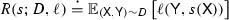, or the $$\ell$$-ranking risk,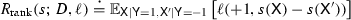.

Given this, the standard problem of learning from binary labels may be stated as:

### Example

We will be interested in two canonical problems of learning from binary labels. In binary classification (Devroye 1996), the goal is to approximately minimise the misclassification error $$R(s; D, \ell ^{01})$$, where $$\ell ^{01}$$ is the zero-one loss $$\ell ^{01}(y,v) =\llbracket yv < 0 \rrbracket + \frac{1}{2} \llbracket v = 0 \rrbracket$$ for indicator function $$\llbracket \cdot \rrbracket$$.

In bipartite ranking (Agarwal and Niyogi 2005), the goal is to approximately minimise the pairwise disagreement $$R_{\mathrm {rank}}( s; D, \ell ^{01})$$, which is also known as one minus the area under the ROC curve (AUROC) of s (Clémençon et al. 2008). The latter is preferred over the misclassification error under class imbalance (Ling and Li 1998).

#### Bayes-optimal scorers and regret

In studying the asymptotic behaviour of learning algorithms, two additional risk-related concepts are useful. A Bayes-optimal scorer is any theoretical risk-minimising scorer $$s^* \in {{\text {argmin }}\, }_{s \in \mathbb {R}^{\mathscr {X}}} \, R( s; D)$$. The regret of a scorer $$s :\mathscr {X}\rightarrow \mathbb {R}$$ is its excess risk over that of any Bayes-optimal scorer,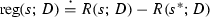.

For example, the set of Bayes-optimal scorers for the misclassification error $$R( \cdot ; D, \ell ^{01})$$ comprises all $$s^*$$ satisfying

\begin{aligned} \mathrm {sign}( s^*( x ) ) = \mathrm {sign}( 2\eta ( x ) - 1 ), \end{aligned}
(1)

so that the sign of an instance’s score matches whether its label is on average positive. Further, the regret for the 0–1 loss is $$\mathrm {reg}( s; D, \ell ^{01}) = \mathbb {E}_{\mathsf {X}\sim M}\left[ | 2\eta ( x ) - 1 | \cdot \llbracket (2 \eta ( \mathsf {X}) - 1) \cdot s( x ) < 0 \rrbracket \right]$$ (Devroye et al. 1996, Theorem 2.2), i.e., the concentration of $$\eta$$ near $$\frac{1}{2}$$ in the region of disagreement with any optimal scorer.

### Learning from corrupted binary labels

Fix some distribution $$D$$. In the problem of learning from corrupted or noisy binary labels, we have a training sample $$\bar{\mathsf {S}}\sim \bar{D}^m$$, for some $$\bar{D}\ne D$$ whose $$\mathbb {P}(\mathsf {X})$$ is unchanged, but $$\mathbb {P}( \bar{\mathsf {Y}}\mid \mathsf {X}= x ) \ne \mathbb {P}( \mathsf {Y}\mid \mathsf {X}= x )$$. That is, we observe samples with the same marginal distribution over instances, but different conditional distribution over labels. Our goal remains to learn a scorer with small risk with respect to $$D$$, despite $$D$$ being unobserved. More precisely, the problem of learning from noisy binary labels may be stated as:

We refer to $$D$$ as the “clean” and $$\bar{D}$$ as the “corrupted” distribution. Note that we allow $$D$$ to be non-separable, i.e., $$\eta ( x ) \in (0, 1)$$ for some $$x \in \mathscr {X}$$; thus, even under $$D$$, there is not necessarily certainty as to every instance’s label. Our use of “noise” and “corruption” thus refers to an additional, exogenous uncertainty in the labelling process.

#### Instance-dependent noise models

We will focus on $$\bar{D}$$ that arise from randomly flipping the labels in $$D$$. Further, our interest is in instance-dependent noise, i.e., noise which depends compulsorily on the instance, and optionally on the label. To capture this, we first introduce the general label- and instance-dependent noise (LIN) model.

### Definition 1

(LIN model) Given a clean distribution $$D$$ and label flip functions $$\rho _1, \rho _{-1} :\mathscr {X}\rightarrow [ 0, 1 ]$$, under the LIN model we observe samples $$( \mathsf {X}, \bar{\mathsf {Y}} ) \sim \bar{D}= \mathrm {LIN}( D, \rho _{-1}, \rho _{1} )$$, where first we draw $$(\mathsf {X}, \mathsf {Y}) \sim D$$ as usual, and then flip $$\mathsf {Y}$$ with probability $$\rho _{\mathsf {Y}}( \mathsf {X})$$ to produce $$\bar{\mathsf {Y}}$$.

The label flip functions $$\rho _{\pm 1}$$ allow one to model label noise with dependences on the instance and true label. We do not impose any parametric assumptions on these functions; the only restriction we place is that on average, the noisy and true labels must agree, i.e.,

\begin{aligned} \sup _{x \in \mathscr {X}} \, \left( \rho _1( x ) + \rho _{-1}( x ) \right) < 1. \end{aligned}
(2)

When $$\rho _{\pm 1}$$ are constant, this is a standard assumption (Blum and Mitchell 1998; Scott et al. 2013). We will refer to $$\rho _{\pm 1}$$ satisfying Eq. 2 as being admissible.

The LIN model may be specialised to the case where the noise depends on the instance, but not the label. We term this the purely instance-dependent noise (PIN) model.

### Definition 2

(PIN model) Given a label flip function $$\rho :\mathscr {X}\rightarrow [0, \nicefrac []{1}{2})$$, under the PIN model we observe samples from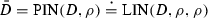.

Both the LIN and PIN models consider noise which is instance-dependent; however, the LIN model is strictly more general. In particular, for non-separable $$D$$, each $$x \in \mathscr {X}$$ has non-zero probability of being paired with either $$\{ \pm 1 \}$$ as a label; thus, under the LIN model, the example $$( x, +1 )$$ occurring in a sample $$\mathsf {S}\sim D^N$$ could have its label flipped with different probability to $$( x, -1 )$$ occurring in another $$\mathsf {S}' \sim D^N$$.

Note that the image of $$\rho$$ in Definition 2 is $$[0, \nicefrac []{1}{2})$$ so as to enforce the condition in Eq. 2. When $$D$$ is separable, this condition is equivalent to enforcing that the noisy class-probabilities are bounded away from $$\frac{1}{2}$$, which is known as a Massart condition (Massart and Nédélec 2006) on the class-probability. Consequently, when $$D$$ is separable, instance-dependent noise satisfying Eq. 2 is also known as a Massart or bounded noise model.

#### Relation to existing models

As a special case, the LIN model captures instance-independent but label-dependent noise. Here, all instances within the same class have the same label flip probability. This is known as the class-conditional noise (CCN) setting, and has received considerable attention (Blum and Mitchell 1998; Natarajan et al. 2013).

### Definition 3

(CCN model) Given label flip probabilities $$\rho _{\pm 1} \in [0, 1]$$, under the CCN model we observe samples from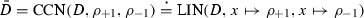.

### Consistency of noisy risk minimisation?

Our primary theoretical interest in learning from LIN or PIN noise is the issue of statistical consistency of noisy risk minimisation. This aims to answer the question: if we can perform near-optimally with respect to some risk on the noisy distribution, will we also perform near-optimally on the clean distribution? More formally, we wish to know if, e.g.,

\begin{aligned} \mathrm {reg}\left( s_n; \bar{D}, \ell ^{01}\right) \rightarrow 0 {\mathop {\implies }\limits ^{?}} \mathrm {reg}\left( s_n; D, \ell ^{01}\right) \rightarrow 0 \end{aligned}
(3)

for any distribution $$D$$, corrupted distribution $$\bar{D}$$, and scorer sequence $$( s_n )_{n = 1}^\infty$$. Establishing this would imply that one can perform near-optimally given sufficiently many noisy samples, and a sufficiently powerful class of scorers. The latter assumption is in keeping with standard consistency analysis for binary classification (Zhang 2004; Bartlett et al. 2006); however, its practical applicability is somewhat limited. To address this, we further study (Sect. 5) an algorithm to efficiently (and provably) learn under instance-dependent noise.

As noted in the Introduction, a number of recent works have established classification consistency of noisy risk minimisation (Scott et al. 2013; Natarajan et al. 2013; Menon et al. 2015) for the special case of class-conditional (and hence instance-independent) noise. A large strand of work has provided PAC-style guarantees under various instance-dependent noise models (Bylander 1997, 1998; Servedio 1999; Awasthi et al. 2015, 2016, 2017). However, these works impose assumptions on both $$D$$ and the class of scorers. For a more detailed comparison and discussion, see Sect. 6.

## Classification consistency under purely instance-dependent noise

We begin with our first contribution (C1), which shows that one can classify optimally given access only to samples corrupted with purely instance-dependent noise, assuming a suitably rich function class and sufficiently many samples; i.e., noisy risk minimisation is consistent.

### Relating clean and corrupt Bayes-optimal scorers

Recall from Eq. 3 that establishing consistency of noisy risk minimisation requires showing that a scorer s that classifies well on the corrupted $$\bar{D}$$ also classifies well on the clean $$D$$, i.e., if the regret $$\mathrm {reg}( s; \bar{D}, \ell )$$ is small for a suitable loss $$\ell$$, then so is $$\mathrm {reg}( s; D, \ell )$$.

Before proceeding, it is prudent to convince ourselves that such a result is possible in the first place. A necessary condition is that the clean and corrupted Bayes-optimal scorers coincide; without this, noisy risk minimisation will converge to the wrong object. For many losses, the Bayes-optimal scorers depend on the underlying class-probability function (c.f. Eq. 1). Thus, to study these scorers on $$\bar{D}$$ resulting from generic label- and instance-dependent noise, we examine its class-probability function $$\bar{\eta }$$.

### Lemma 1

Pick any distribution $$D$$. Suppose $$\bar{D}= \mathrm {LIN}( D, \rho _{-1}, \rho _{1} )$$ for admissible label flip functions $$\rho _{\pm 1} :\mathscr {X}\rightarrow [ 0, 1 ]$$. Then, $$\bar{D}$$ has corrupted class-probability function

\begin{aligned} \left( \forall x \in \mathscr {X}\right) \, \bar{\eta }( x ) = \left( 1 - \rho _1( x )\right) \cdot \eta ( x ) + \rho _{-1}( x ) \cdot \left( 1 - \eta ( x )\right) . \end{aligned}
(4)

The form of Eq. 4 is intuitive: the corrupted positives can be seen as a mixture of a positive and negative instances, with mixing weights determined by the flip probabilities. This also illustrates that the effect of noise is to compress the range of $$\eta$$, thus increasing one’s uncertainty as to an instance’s label.

Lemma 1 implies that we cannot hope to establish consistency without further assumptions. For example, with the 0–1 loss, Eq. 1 established that any Bayes-optimal scorer $$s^*$$ on $$D$$ has $$\mathrm {sign}( s^*( x ) ) = \mathrm {sign}( \eta ( x ) - \nicefrac []{1}{2})$$. However, if $$\rho _{1}$$ and $$\rho _{-1}$$ vary arbitrarily, then it is easy to check from Eq. 4 that the $$\mathrm {sign}( \eta ( x ) - \nicefrac []{1}{2}) \ne \mathrm {sign}( \bar{\eta }( x ) - \nicefrac []{1}{2})$$. Consequently, the clean and corrupted optimal scorers will differ, and we will not have consistency in general.

Fortunately, we can make progress under two further assumptions: that the noise is purely instance-dependent (per Definition 2), and following (Ghosh et al. 2015), that

\begin{aligned} ( \forall v \in \mathbb {R}) \, \ell ( +1, v ) + \ell ( -1, v ) = C \end{aligned}
(5)

for some $$C \in \mathbb {R}$$. Equation 5 holds for the zero-one, ramp, and “unhinged” loss (van Rooyen et al. 2015). Under these restrictions, the clean and corrupted optimal scorers agree.

### Corollary 1

Pick any distribution $$D$$, and loss $$\ell$$ satisfying Eq. 5. Suppose that $$\bar{D}= \mathrm {PIN}( D, \rho )$$ for admissible label flip function $$\rho :\mathscr {X}\rightarrow [0, \nicefrac []{1}{2})$$. Then,

\begin{aligned} \underset{s \in \mathbb {R}^{\mathscr {X}}}{{\text {argmin }}\, } R( s; D, \ell ) = \underset{s \in \mathbb {R}^{\mathscr {X}}}{{\text {argmin }}\, } R( s; \bar{D}, \ell ). \end{aligned}

For the case of 0–1 loss, Corollary 1 is intuitive: with purely instance-dependent noise satisfying the condition in Eq. 2, the corrupted label will agree on average with the true label; thus, the Bayes-optimal classifier, which simply looks at whether an instance is more likely on average to be positive or negative, will remain the same.

We emphasise that Corollary 1 does not require $$D$$ to have a deterministic labelling function, i.e., it does not require separability of the distribution. Corollary 1 generalises Natarajan et al. (2013, Corollary 10), which was for instance-independent noise. Awasthi et al. (2015), Ghosh et al. (2015, Theorem 1) made a similar observation, but only for 0–1 loss and under the additional assumption of $$D$$ being separable, i.e., $$\eta ( x ) \in \{ 0, 1 \}$$.

### Relating clean and corrupt regrets

Having established the equivalence of the clean and corrupted optimal scorers, the next step in showing consistency is relating the clean and the corrupted regrets. We have the following, which relies on the same assumptions on the noise and loss as Corollary 1.

### Theorem 1

Pick any distribution $$D$$, and loss $$\ell$$ satisfying Eq. 5. Suppose $$\bar{D}= \mathrm {PIN}( D, \rho )$$ for admissible label flip function $$\rho :\mathscr {X}\rightarrow [0, \nicefrac []{1}{2})$$. Then, for any $$s :\mathscr {X}\rightarrow \mathbb {R}$$,

\begin{aligned} \mathrm {reg}\left( s; D, \ell \right) \le \left( {1 - 2 \cdot \rho _{\mathrm {max}}}\right) ^{-1} \cdot \mathrm {reg}\left( s; \bar{D}, \ell \right) \end{aligned}
(6)

where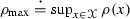. Further, if $$\sup _{y, v} | \ell ( y, v ) | = B < +\infty$$, then for any $$\alpha \in [0, 1]$$,

\begin{aligned} \begin{aligned} \mathrm {reg}\left( s; D, \ell \right) \le&\left( ( 1 - 2 \cdot \rho _{\mathrm {max}} )^{-1} \cdot \mathrm {reg}\left( s; \bar{D}, \ell \right) \right) ^{1 - \alpha } \cdot \left( B \cdot \mathbb {E}_{\mathsf {X}\sim M}\left[ (1 - 2 \cdot \rho ( \mathsf {X}))^{-1} \right] \right) ^\alpha . \end{aligned} \end{aligned}
(7)

The proof of Theorem 1 relies on the observation that the clean risk can be written as a weighted corrupted risk. We thus simply bound these weights, and appeal to the fact that the clean and corrupted regrets both involve the same Bayes-optimal scorer (Corollary 1).

#### Implications

For the zero-one loss $$\ell ^{01}$$, Theorem 1 implies that for a sequence of scorers $$( s_n )_{n = 1}^\infty$$, if $$\mathrm {reg}( s_n; \bar{D}, \ell ^{01}) \rightarrow 0$$, then $$\mathrm {reg}( s_n; D, \ell ^{01}) \rightarrow 0$$ as well; i.e., consistent classification on the corrupted distribution implies consistency on the clean distribution as well. Thus, with powerful models and sufficient data, we can optimally classify even when learning solely from noisy labels. One can achieve $$\mathrm {reg}( s; \bar{D}, \ell ^{01}) \rightarrow 0$$ by minimising any appropriate convex surrogate to $$\ell ^{01}$$ on $$\bar{D}$$ (e.g. hinge, logistic, exponential), owing to standard classification calibration results (Zhang 2004; Bartlett et al. 2006). Importantly, this surrogate does not have to satisfy Eq. 5.

In Eq. 7, $$\alpha$$ may be chosen (in a distribution-dependent manner) to yield the tightest possible bound. When $$\alpha = 0$$, the bound is identical to Eq. 6. However, when $$\alpha > 0$$, the former explicates how the regret depends on the average noise rate of instances, while the latter pessimistically focusses on the maximal noise rate. In particular, Eq. 7 illustrates that when most instances have low noise ($$\rho ( x ) \sim 0$$), one is not overly harmed by a small fraction of instances with high noise: even if $$\rho _{\mathrm {max}} \sim \nicefrac []{1}{2}$$, the second term term will dominate and the regret on the clean distribution will be small. At the other extreme, when $$\rho ( x ) \sim \nicefrac []{1}{2}$$ for most x, while we still have asymptotic consistency, there will be a large relative difference in the clean and absolute regrets. This is also as expected, since the presence of noise intuitively must make the learning task more challenging.

#### Extensions

The regret bound in Theorem 1 may be combined with standard surrogate regret and generalisation bounds applied to the noisy risk minimisation problem. Specifically, per the bounds of Bartlett et al. (2006), Eq. 6 can be further bounded as

\begin{aligned} \mathrm {reg}\left( s; D, \ell ^{01}\right) \le ({1 - 2 \cdot \rho _{\mathrm {max}}})^{-1} \cdot \Psi \left( \mathrm {reg}\left( s; \bar{D}, \ell \right) \right) \end{aligned}
(8)

where $$\ell$$ is a classification-calibrated loss, and $$\Psi$$ the corresponding calibration function as per (Bartlett et al. 2006, Definition 2). For example, $$\Psi ( z ) = z$$ for the hinge loss $$\ell ^{\mathrm {hng}}$$.

We may further specify how the regret on $$D$$ decays given a scorer derived from a finite noisy sample with a suitable function class, by combining Eq. 8 with results on the behaviour of $$\mathrm {reg}( s; \bar{D}, \ell )$$. Formally, given a noisy sample $$\bar{\mathsf {S}} \sim \bar{D}^n$$, let $$\bar{s}_n$$ denote the regularised empirical minimiser of the hinge loss $$\ell ^{\mathrm {hng}}$$ over a kernelised scorer class $$\mathscr {S}= \{ x \mapsto \langle w, \Phi ( x ) \rangle _{\mathscr {H}} \}$$, for feature mapping $$\Phi :\mathscr {X}\rightarrow \mathscr {H}$$ and reproducing kernel Hilbert space $$\mathscr {H}$$. Then, with probability at least $$1 - \delta$$, (Steinwart and Scovel 2005, Theorem 1)

\begin{aligned} \mathrm {reg}\left( \bar{s}_n; \bar{D}, \ell ^{\mathrm {hng}}\right) = \mathscr {O}\left( \left( \log \frac{1}{\delta } \right) ^2 \cdot \frac{1}{n^{\alpha \cdot \beta }} \right) \end{aligned}
(9)

where $$\alpha$$ is such that the strength of regularisation is $$\lambda _n = {n^{-\alpha }}$$, and $$\beta$$ controls the approximation error from using kernelised (rather than all measurable) scorers.

#### Related work

Theorem 1 generalises Natarajan et al. (2013, Theorem 11), which was for instance-independent noise. Ghosh et al. (2015, Theorem 1) provided a distinct bound between clean and corrupted risks, which does not establish consistency. Awasthi et al. (2015, 2016) established small corrupted 0–1 regret for specific algorithms under separable $$D$$, while our bound relates clean and corrupted regrets for the output of any algorithm. See also Sect. 6.

### Beyond misclassification error?

Theorem 1 implies consistency for the misclassification error. In practice, other measures such as the balanced error and F-score are also practically pervasive, especially under class imbalance. Can we show consistency for such measures as well?

Disappointingly, the answer is no. The reason is simple: for a range of such classification measures, any optimal scorer on $$D$$ has $$\mathrm {sign}( s^*( x ) ) = \mathrm {sign}( \eta ( x ) - t(D) )$$, where $$t(D)$$ is some possibly distributional dependent threshold (Narasimhan et al. 2014; Koyejo et al. 2014). However, Eq. 4 reveals that retaining such an optimal scorer on $$\bar{D}$$ is not possible, as

\begin{aligned} ( \forall x \in \mathscr {X}) \, \eta ( x )> t \iff \bar{\eta }( x ) > t + \rho ( x ) \cdot (1 - 2 \cdot t); \end{aligned}

i.e., the thresholds of the clean and corrupted class-probability function do not coincide in general, so that no analogue of Corollary 1 can possibly hold. Specifically, for any $$t \ne \nicefrac []{1}{2}$$ (i.e. any threshold beyond that for 0–1 loss), optimal classification based on $$\bar{\eta }$$ requires knowing the unknown flipping function $$\rho ( x )$$.

The above implies that under purely instance-dependent noise, we cannot (at least naïvely) optimally classify with measures beyond the misclassification error. This is a point of departure from existing analysis for instance-independent noise; for example, Menon et al. (2015) established that the balanced error minimiser is unaffected under class-conditional noise.

## AUROC consistency under boundary-consistent noise

Having established classification consistency for purely instance-dependent noise, we turn to our second contribution (C2), concerning the distinct problem of bipartite ranking consistency. Recall from Sect. 2.1 that bipartite ranking (Agarwal and Niyogi 2005) considers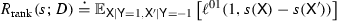viz. one minus the area under the ROC curve (AUROC) of s (Clémençon et al. 2008).

Given the popularity of the AUROC as a performance measure under class imbalance (Ling and Li 1998), studying its consistency under label noise is of interest. However, compared to the misclassification error, even in the instance-independent case, this issue has received comparatively little attention, with a few exceptions (Menon et al. 2015). We now provide such an analysis for a structured form of label- and instance-dependent noise.

### Relating clean and corrupt Bayes-optimal scorers

As in Sect. 3, before studying AUROC consistency, it is prudent to confirm that the clean and corrupted Bayes-optimal scorers of the AUROC coincide. The AUROC is maximised by any scorer $$s^*$$ that is order preserving for $$\eta$$ (Clémençon et al. 2008), i.e.

\begin{aligned} ( \forall x, x' \in \mathscr {X}) \, \eta ( x )< \eta ( x' ) \implies s^*( x ) < s^*( x' ). \end{aligned}

Equally, on the corrupted $$\bar{D}$$, the corrupted AUROC will be maximised by any scorer that is order preserving for $$\bar{\eta }$$. Thus, for the Bayes-optimal scorers to coincide, we will have to ensure that $$\bar{\eta }$$ is order preserving for $$\eta$$, i.e., that

\begin{aligned} ( \forall x, x' \in \mathscr {X}) \, \eta ( x )< \eta ( x' ) \implies \bar{\eta }( x ) < \bar{\eta }( x' ). \end{aligned}
(10)

But by Lemma 1, this cannot be true for general label- and instance-dependent noise, since there is no necessary relationship between the flip functions $$\rho _{\pm 1}$$ and $$\eta$$; see “Appendix C” for some concrete counter-examples.

To make progress, we thus need to restrict our noise model by injecting suitable dependence between $$\rho _{\pm 1}$$ and $$\eta$$. We next present one such noise model which suits our needs.

### The boundary consistent noise (BCN) model

We propose a noise model where, roughly, the higher the inherent uncertainty (i.e., $$\eta \approx \nicefrac []{1}{2}$$), the higher the noise. We will shortly show such a model possesses order preservation.

### Definition 4

(BCN model) Given a clean distribution $$D$$, consider an label- and instance-dependent noise model $$\mathrm {LIN}( D, \rho _{-1}, \rho _{1} )$$ where $$\rho _y = f_y \circ s$$ for some functions $$f_{\pm 1} :\mathbb {R}\rightarrow [0, 1]$$ and $$s :\mathscr {X}\rightarrow \mathbb {R}$$ such that:

1. (a)

s is order preserving for $$\eta$$ i.e., $$( \forall x, x' \in \mathscr {X}) \, \eta ( x )< \eta ( x' ) \implies s( x ) < s( x' ).$$

2. (b)

$$f_{\pm 1}$$ are non-decreasing on $$(-\infty , s_0]$$ and non-increasing on $$[s_0, \infty )$$, where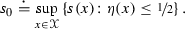3. (c)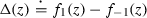is non-increasing.

We term this the boundary consistent noise model (BCN model). We write the resulting corrupted distribution as $$\mathrm {BCN}( D, f_{-1}, f_{1}, s )$$.

The $$\mathrm {BCN}$$ noise model is, to our knowledge, novel. However, special cases of the model have been studied by Bylander (1997), Du and Cai (2015) and Bootkrajang (2016), wherein it is assumed that $$D$$ is linearly separable, and the noise is purely instance-dependent. As one such special case, the BCN model captures a plausible model of human annotator noise, wherein “hard” instances (i.e. those close to some optimal separator) have the most noise.

### Example 1

(Annotator noise) Suppose $$s( x ) = \langle w^*, x \rangle$$ for some $$w^* \in \mathbb {R}^d$$. Consider a linearly separable $$D$$ with $$\eta ( x ) = \llbracket s( x ) > 0 \rrbracket$$, and noise $$\mathrm {LIN}( D, \rho _{-1}, \rho _{1} )$$ where $$\rho _{-1} = \rho _{1} = f \circ s$$, and $$f_{\pm 1}( z ) = g(| z |)$$ for some monotone decreasing g.

We now unpack the three conditions underpinning the general model:

1. (a)

encodes that the scores underlying the noise order instances consistently with $$\eta$$.

2. (b)

encodes that “harder” instances (with $$\eta \approx \nicefrac []{1}{2}$$) have the highest chance of a label flip.

3. (c)

is more opaque; however, it is trivially satisfied when the flip functions are constant (i.e., the noise is class-conditional), or identical (i.e. the noise is purely instance-dependent). The latter covers the practically relevant Example 1; thus, all results for $$\mathrm {BCN}$$ automatically hold for this important case. In more general settings, the condition is needed for technical reasons (see Sect. 4.3 and “Appendix C”).

### Relating clean and corrupt regrets

We now show that under the $$\mathrm {BCN}$$ model, order preservation of $$\eta$$ is guaranteed as per Eq. 18. Thus, the clean and corrupt Bayes-optimal AUROC scorers coincide.

### Proposition 1

Pick any distribution $$D$$. Suppose $$\bar{D}= \mathrm {BCN}( D, f_{-1}, f_{1}, s )$$. Then,

\begin{aligned} \left( \forall x, x' \in \mathscr {X}\right) \, \eta ( x )< \eta ( x' ) \implies \bar{\eta }( x ) < \bar{\eta }( x' ). \end{aligned}

While simple to state, the result requires a careful analysis of the relationship between $$\bar{\eta }( x ) - \bar{\eta }( x' )$$ and $$\eta ( x ) - \eta ( x' )$$. Further, it crucially requires Condition (c) of the $$\mathrm {BCN}$$ model; see “Appendix C” for counterexamples, including one where $$f_1( z ) - f_{-1}( z )$$ is non-decreasing rather than non-increasing.

Proposition 1 reassures us that under the $$\mathrm {BCN}$$ model, corrupted ranking risk minimisation converges to the right object. A careful analysis of the behaviour of $$(\bar{\eta }( x ) - \bar{\eta }( x' ))/(\eta ( x ) - \eta ( x' ))$$ lets us go further and provide a ranking regret bound, analogous to Theorem 1.

### Theorem 2

Pick any distribution $$D$$. Let $$\bar{D}$$ be a corrupted distribution such that $$(\eta , \bar{\eta })$$ satisfy Eq. 10, and there exists a constant C such that

\begin{aligned} \left( \forall x, x' \in \mathscr {X}\right) \, | \eta ( x ) - \eta ( x' ) | \le C \cdot | \bar{\eta }( x ) - \bar{\eta }( x' ) |. \end{aligned}
(11)

Then, for any scorer $$s :\mathscr {X}\rightarrow \mathbb {R}$$,

\begin{aligned} \mathrm {reg}_{\mathrm {rank}}( s; D) \le \, C \cdot ( {\pi \cdot (1 - \pi )} )^{-1} \cdot \,{\bar{\pi }\cdot (1 - \bar{\pi })} \cdot \mathrm {reg}_{\mathrm {rank}}( s; \bar{D}) \end{aligned}
(12)

where $$\mathrm {reg}_{\mathrm {rank}}$$ denotes the excess ranking risk of a scorer s, and $$\pi = \mathbb {P}( \mathsf {Y}= 1 )$$, $$\bar{\pi }= \mathbb {P}( \bar{\mathsf {Y}}= 1)$$.

In particular, if $$\bar{D}= \mathrm {BCN}( D, f_{-1}, f_{1}, s )$$ where $$( f_{-1}, f_{1}, s, \eta )$$ are $$\mathrm {BCN}$$-admissible, and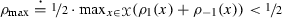, then Eq. 12 holds with $$C = ({1 - 2 \cdot \rho _{\mathrm {max}})}^{-1}$$.

Intuitively, the condition in Eq. 11 ensures that if a pair of instances are easy to distinguish on the clean distribution (e.g., $$\eta ( x ) = 1$$ while $$\eta ( x' ) = 0$$), they remain relatively so on the corrupted distribution. This rules out scenarios where the noise makes all instances, regardless of their original $$\eta$$ value, have an $$\bar{\eta }$$ value arbitrarily close to $$\nicefrac []{1}{2}$$.

#### Implications

Theorem 2 implies that, under BCN  noise, we can optimally rank (in the sense of AUROC) even when learning solely from noisy labels. Note that we can make $$\mathrm {reg}_{\mathrm {rank}}( s; \bar{D}) \rightarrow 0$$ by appropriate surrogate loss minimisation on $$\bar{D}$$ (Agarwal 2014).

Note also that neither of the noise models in Theorems 1 and 2 are special cases of each other. In particular, Theorem 2 allows for the noise to depend on the label, while Theorem 1 does not. However, even under purely instance-dependent noise, Theorem 2 requires the flip function $$\rho$$ to satisfy additional conditions so as to guarantee order-preservation.

As a final remark, we note that the BCN model is only sufficient for establishing Theorem 2: as stated, the necessary conditions are that $$\bar{\eta }$$ is order-preserving for $$\eta$$, and there is a bound on the ratio $$({\bar{\eta }( x ) - \bar{\eta }( x' )})/({\eta ( x ) - \eta ( x' )})$$. We focus on BCN as it is a plausible model of real-world noise, and leave for future work the exploration of other admissible noise models.

#### Related work

Theorem 2 generalises Menon et al. (2015, Corollary 3), which assumed instance-independent noise. This generalisation is non-trivial, with the proof of Proposition 1 requiring a careful case-based analysis. We are not aware of any prior analysis of the consistency of AUROC maximisation under noise with any form of instance-dependence.

## The Isotron: efficiently learning under boundary-consistent noise

Theorems 1 and 2 imply that by ensuring vanishing regret on the corrupted distribution, we also ensure vanishing regret on the clean distribution. We now turn to our third contribution (C3), concerning the algorithmic implications of our results, by specifying how precisely one can minimise the corrupted regret in practice.

A standard approach is to choose s from a rich function class, e.g., that of a universal kernel with appropriately tuned parameters. However, this is potentially unsatisfying in two ways. First, training a kernel machine without further approximation requires quadratic complexity (Schölkopf 2001, p. 288), which may be computationally infeasible. Second, suppose one has further knowledge about the clean $$D$$, e.g., that it is well-modelled by a linear scorer in the native feature space. Employing a generic kernel machine here is intuitively overkill, and does not exploit our prior knowledge. As a practical consequence, we expect such an approach to generalise worse than one that directly uses a linear model.

We now show that, when we know the clean $$D$$ can be modelled by a linear scorer (allowing but not requiring $$D$$ to be linearly separable), the Isotron algorithm (Kalai and Sastry 2009) can provably and efficiently learn under certain boundary-consistent noise. To make this more precise, we need to introduce two additional concepts.

### The SIM family of class-probability functions

Our assumption on $$D$$ will be that it belongs to some member of the generalised linear model (GLM) family. More formally, for link function $$u :\mathbb {R}\rightarrow [0, 1]$$ and separator $$w^* \in \mathbb {R}^d$$, the GLM class-probability function is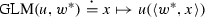. We assume $$D$$ belongs to the single-index model (SIM) family of class-probability functions (Kalai and Sastry 2009), wherein the link is unknown, but is known to be Lipschitz. That is, the SIM family comprises all possible GLM models with Lipschitz link.

### Definition 5

(SIM family) For any $$L, W \in \mathbb {R}_+$$, the single-index model (SIM) family is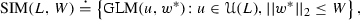where $$\mathscr {U}( L )$$ is all non-decreasing L-Lipschitz functions.

Intuitively, the SIM assumption on $$D$$ encodes that a linear model equipped with a suitable non-linearity can accurately predict the labels. Two simple examples are presented below.

### Example 2

Suppose that $$D$$ is linearly separable with margin $$\gamma > 0$$, i.e., $$\eta ( x ) = \llbracket \langle w^*, x \rangle > 0 \rrbracket$$ where $$\mathbb {P}( \{ (\mathsf {X}, \mathsf {Y}) \mid \mathsf {Y}\cdot \langle w^*, \mathsf {X}\rangle < \gamma \} ) = 0.$$ Then, $$\eta \in \mathrm {SIM}( (2\gamma )^{-1}, || w^* || )$$ (Kalai and Sastry 2009). This is since we can equally write $$\eta ( x ) = u_{\mathrm {mar}( \gamma )}( \langle w^*, x \rangle )$$, where

\begin{aligned} u_{\mathrm {mar}( \gamma )}( z )&= {\left\{ \begin{array}{ll} 1 &{}\quad \text { if } z > \gamma \\ \frac{z + \gamma }{2 \gamma } &{}\quad \text { if } z \in [-\gamma , +\gamma ]\\ 0 &{}\quad \text { if } z < -\gamma . \end{array}\right. } \end{aligned}
(13)

The function $$u( \cdot )$$ is clearly $$(2\gamma )^{-1}$$-Lipschitz.

### Example 3

Suppose that $$D$$ has class-probability of the logistic regression form, i.e., $$\eta ( x ) = ({1 + e^{-\langle w^*, x \rangle }})^{-1}.$$ Then, $$\eta \in \mathrm {SIM}( 1, || w^* || )$$.

### The SIN family of noise models

Our assumption on the noise will be that the distance from the optimal separator determines the level of noise. More formally, suppose our clean $$D$$ has $$\eta = \mathrm {GLM}( u, w^* )$$ for some (unknown) $$u, w^*$$. We then consider a boundary consistent model of the noise with $$s^*( x ) = \langle w^*, x \rangle$$ determining the flip probabilityFootnote 1; we shall call this the single index noise (SIN) model.

### Definition 6

(SIN noise) Let $$f_1, f_{-1} :\mathbb {R}\rightarrow [ 0, 1 ]$$. Given any distribution $$D$$ with $$\eta = \mathrm {GLM}( u, w^* )$$, define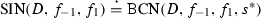where $$s^* :x \mapsto \langle w^*, x \rangle$$.

We shall see concrete examples of this noise model shortly. Put simply, like the underlying boundary-consistent noise model, it posits that inherently “hard” instances experience the most noise. To see this, suppose $$D$$ is linearly separable. Then, instances close to $$w^*$$ are “hard” in the sense that they are optimally classified with low confidence; intuitively, such instances are easily confusable with instances from the other class.

### Corruption runs in the SIN family

Under the SIM assumption on $$D$$ and SIN assumption on the noise, learning from the resulting corrupted distribution $$\bar{D}$$ is non-trivial: even if we know the correct link function $$u( \cdot )$$ for $$D$$, we will not know the precise link under $$\bar{D}$$, as this will be affected by the (unknown) noise. Thus, we cannot directly leverage a standard GLM to provably learn from $$\bar{D}$$.

Fortunately, an appealing consequence of pairing the SIM and SIN assumptions is that the SIM family is closed under SIN corruption, i.e., the resulting corrupted distribution is also a member of the SIM family.

### Proposition 2

Pick any distribution $$D$$ with $$\eta \in \mathrm {SIM}( L, W )$$. Suppose that $$\bar{D}= \mathrm {SIN}( D, f_{-1}, f_{1} )$$ where $$( f_{-1}, f_{1}, \eta )$$ are $$\mathrm {BCN}$$-admissible, and $$( f_{-1}, f_{1} )$$ are $$( L_{-1}, L_{1} )$$-Lipschitz respectively. Then, $$\bar{\eta }\in \mathrm {SIM}( L + L_{-1} + L_{1}, W )$$. In particular, $$\bar{\eta }( x ) = \bar{u}( \langle w^*, x \rangle )$$ where

\begin{aligned} \bar{u}( z )&= ( 1 - f_1( z ) ) \cdot u( z ) + f_{-1}( z ) \cdot ( 1 - u ( z ) ). \end{aligned}
(14)

This result is intuitive in light of Proposition 1, as $$\bar{\eta }$$ is order preserving for $$\eta$$ under $$\mathrm {BCN}$$. To illustrate this further, we provide two examples of corrupting the SIM member $$\eta ( x ) = u( \langle w^*, x \rangle )$$ by SIN noise.

### Example 4

Consider the class-conditional noise regime, so that $$f_1 \equiv \rho _{+}, f_{-1} \equiv \rho _{-}$$ for constants $$\rho _{\pm } \in [0, 1]$$. Then, by Eq. 4, $$\bar{\eta }( x ) = \bar{u}( \langle w^*, x \rangle )$$ for $$\bar{u}( z ) = ( 1 - \rho _{+} - \rho _{-} ) \cdot u( z ) + \rho _{-}.$$

### Example 5

Suppose $$f_1 \equiv f_{-1} \equiv f$$ and $$f( z ) = g( | z | )$$ for some arbitrary monotone decreasing function g. Then, by Eq. 4, $$\bar{\eta }( x ) = \bar{u}( \langle w^*, x \rangle )$$ for $$\bar{u}( z ) = ( 1 - 2 \cdot f( z) ) \cdot u( z ) + f( z ).$$ If we further assume $$u( z ) = \llbracket z > 0 \rrbracket$$, so that $$D$$ is separable, we have

\begin{aligned} \bar{u}( z )&= {\left\{ \begin{array}{ll} 1 - g( z ) &{}\quad \text { if } z > 0 \\ g( -z ) &{}\quad \text { if } z < 0. \end{array}\right. } \end{aligned}

Observe that if g satisfies $$g( -z ) = 1 - g( z )$$, then this is $$\bar{u}( z ) = g( -z ).$$ That is, a structured form of monotonic noise on a linearly separable distribution yields a distribution scorable by some generalised linear model. When $$g( z ) = {1}/({1 + e^{z}})$$ for example, we end up with a logistic regression model. This observation has been made previously (Du and Cai 2015).

We are not aware of prior results akin to Proposition 2 on the behaviour of SIMs under structured noise. However, when $$D$$ is separable, Du and Cai (2015) observed that a certain special case of our $$\mathrm {BCN}$$ noise results in an $$\bar{\eta }$$ that belongs to the GLM family.

Proposition 2 implies that any algorithm for learning a generic SIM $$D$$ may be used to learn $$\bar{\eta }$$ under SIN noise. Fortunately, we now see efficient algorithms to learn SIMs exist.

### Efficiently learning noisy SIMs via the Isotron

SIMs for instances in the unit ball $$\mathbb {B}^d$$ can be provably learned with the Isotron (Kalai and Sastry 2009), and its Lipschitz variant, the SLIsotron (Kakade et al. 2011). The elegant Isotron algorithm (Algorithm 1) alternately updates the separator w, and the link function u. The latter is estimated non-parametrically using the pav algorithm (Ayer et al. 1955), which solves the isotonic regression problem: $$( \hat{{u}}_1, \ldots , \hat{{u}}_m ) = {{\text {argmin }}\, }_{{u}_1 \le {u}_2 \le \cdots \le {u}_m}{\sum _{i = 1}^m ( y_i - {u}_i )^2},$$ where we pre-sort the scores so that $$s_1 \le s_2 \le \cdots \le s_m$$, i.e., we wish for the u’s to respect the ordering of the s’s. The SLIsotron algorithm is identical, except that one calls lpav, a variant of pav that obeys a Lipschitz constraint.

In light of Proposition 2, we thus propose to simply run the SLIsotron on corrupted samples. One can guarantee ranking consistency of this procedure; further, if the noise does not depend on the label, then we also have classification consistency.

### Theorem 3

Let $$\mathscr {X}\subseteq \mathbb {B}^d$$. Pick any distribution $$D$$ with $$\eta \in \mathrm {SIM}(L, W)$$, and $$\bar{D}= \mathrm {SIN}( D, f_{-1}, f_{1} )$$ for Lipschitz $$( f_{-1}, f_{1} )$$. Given a corrupted sample $$\bar{\mathsf {S}} \sim \bar{D}^n$$, we can construct a corrupted class-probability estimator $$\hat{\bar{\eta }}_{\bar{\mathsf {S}}} :\mathscr {X}\rightarrow [0, 1]$$ using the SLIsotron, with $$\mathrm {reg}_{\mathrm {rank}}( \hat{\bar{\eta }}_{\bar{\mathsf {S}}}; D) {\mathop {\rightarrow }\limits ^{\mathbb {P}}} 0.$$ Further, if $$f_{-1} = f_{1}$$, we can construct a classifier $$c_{\bar{\mathsf {S}}} :x \mapsto \mathrm {sign}( 2\hat{\bar{\eta }}_{\bar{\mathsf {S}}}( x ) - 1 )$$ with $$\mathrm {reg}( c_{\bar{\mathsf {S}}}; D, \ell ^{01}) {\mathop {\rightarrow }\limits ^{\mathbb {P}}} 0.$$

Intuitively, Theorem 3 relies on the existing SLIsotron consistency guarantee for its class-probability estimate (see “Appendix B.5” for a review). Since the SLIsotron is applied on corrupted samples, this implies a suitable corrupted regret asymptotically vanishes. Combined with our classification and ranking regret bounds (Theorems 1 and 2), this implies the clean regret for this estimator also asymptotically vanishes.

#### Implications

We make some additional remarks on the use of the SLIsotron under label noise. First, the SLIsotron does not require one to know the precise form of either $$\eta$$ or the label flipping functions. Even if one just knows that there exists some u such that $$\eta = \mathrm {GLM}(u,w^*)$$, and that the labels are subject to (Lipschitz) monotonic noise, one can estimate $$\bar{\eta }$$.

Second, by estimating $$\bar{\eta }$$, one can potentially estimate the flipping functions themselves. For example, in the class-conditional setting, we can estimate the label flip probabilities via the range of $$\bar{\eta }$$, under a mild assumption on $$D$$ (Scott et al. 2013; Liu and Tao 2015; Menon et al. 2015). For SIN noise, estimation is possible if one knows the precise form of $$u( \cdot )$$, and if the noise does not depend on the labels. For example, one may know that $$D$$ is separable with a certain margin. Then, we can infer the label flipping function as

\begin{aligned} f( z ) = \frac{\bar{u}( z ) - u( z )}{1 - 2 \cdot u( z )}. \end{aligned}

The estimation error in this term depends wholly on the error in estimating $$\bar{u}$$.

Third, while Theorem 3 is a statement about asymptotic consistency, one can establish rates of convergence as well. For example, the SLIsotron guarantee is that the regret of the corrupted class-probability estimates decays like $$\mathscr {O}\left( ( {d}/{n} )^{1/3} \right)$$ (see “Appendix B.5” for a review). This can be contrasted to the regret decay for kernelised scorers (Eq. 9), which can be significantly larger in the regime of low regularisation (which is to expected for low-dimensional problems). This makes concrete our motivating intuition for the potential limitation of using a black-box kernel machine to tackle problems with additional structure.

#### Related work

Existing analysis of the Isotron has focussed on the setting of standard learning from binary labels (Kalai and Sastry 2009; Kakade et al. 2011); to our knowledge, there is no existing analysis of its behaviour under label noise.

Recently, Awasthi et al. (2015, 2016) proposed efficient algorithms to learn under purely instance-dependent noise (PIN), assuming that $$D$$ is linearly separable with log-concave isotropic marginal over instances. Our use of the Isotron operates with a more structured form of noise (SIN), which is a subset of PIN; however, we do not require an assumption on the marginals, and merely require $$D$$ to be linearly scorable by belonging in the GLM family. Further, we show ranking as well as classification consistency.

To learn under class-conditional noise with linear models, Natarajan et al. (2013) proposed a loss-correction requiring knowledge of the noise rates, and Menon et al. (2015) proposed a neural network. The Isotron is distinct from the former by not requiring the noise to be known; from the latter by having a correctness guarantee; and from both by working for noise that can depend on the instances.

## Related work

Recall that our three contributions C1C3 are in showing the classification and ranking consistency of risk minimisation under suitably constrained instance-dependent noise, and a practical algorithm that can learn from such data. We now detail how these contributions are distinct from a number of existing works in label noise. Table 2 provides a summary.

### Three strands of label noise research

While there is too large a body of work on label noise to summarise here (see e.g. Frénay and Kabán 2014; Frénay and Verleysen 2014 for recent surveys), broadly, there have been three strands of theoretical analysis S1–S3 that are relevant to our work.

1. (S1)

PAC guarantees The first strand has focussed on PAC-style guarantees for learning under symmetric and class-conditional noise (e.g. Bylander 1994; Blum et al. 1996; Blum and Mitchell 1998), noise consistent with the distance to the margin (e.g. Angluin and Laird 1988; Bylander 1997, 1998; Servedio 1999), noise constant on partitions of the input space (e.g. Decatur 1997; Ralaivola et al. 2006), noise with bounded error rate (e.g. Kalai et al. 2005; Awasthi et al. 2014), and arbitrary bounded instance dependent or Massart noise (e.g. Awasthi et al. 2015). These works often assume the true distribution $$D$$ is linearly separable with some margin, the marginal over instances has some structure (e.g. uniform over the unit sphere, or log-concave isotropic), and that one employs linear scorers for learning.

2. (S2)

Surrogate losses The second strand has focussed on the design of surrogate losses robust to label noise. Stempfel and Ralaivola (2009) proposed a non-convex variant of the hinge loss robust to asymmetric noise; however, it requires knowledge of the noise rate. For class-conditional noise, Natarajan et al. (2013) provided a simple “noise-corrected” version of any loss, which again requires knowledge of the noise rate. Ghosh et al. (2015) showed that losses whose components sum to a constant are robust to symmetric label noise. van Rooyen et al. (2015) showed that the linear or unhinged loss is robust to symmetric label noise. Patrini et al. (2016) showed that a range of “linear-odd” losses are approximately robust to asymmetric noise.

3. (S3)

Consistency The third strand, which is closest to our work, has focussed on showing consistency of appropriate risk minimisation in the regime where one has a suitably powerful function class (Scott et al. 2013; Natarajan et al. 2013; Menon et al. 2015). For example, Natarajan et al. (2013) showed that minimisation of appropriately weighted convex surrogates on the corrupted distribution $$\bar{D}$$ is consistent for the purposes of classification on $$D$$. This work has been restricted to the case of symmetric- and class-conditional noise.

The difference of the present paper to these works may be summarised as:

1. (a)

we work with instance-dependent noise models (unlike S2 and S3); this is more practically relevant than the standard instance-independent noise assumption.

2. (b)

we do not make assumptions on $$D$$ for our theoretical analysis in Sects. 3 and 4 (unlike S1); this is in keeping with standard consistency results for binary classification (Zhang 2004; Bartlett et al. 2006).

3. (c)

we do not assume the scorer class is linear, but rather that it is sufficiently powerful to contain the Bayes-optimal scorer (unlike S1 and S2); this is again in keeping with consistency results for binary classification (Zhang 2004; Bartlett et al. 2006).

4. (d)

we study consistency with respect to the AUROC, unlike all works (to our knowledge) with the exception of Menon et al. (2015); this is of interest since the AUROC is a canonical performance measure under class imbalance (Ling and Li 1998).

5. (e)

we explicitly provide a practical algorithm for learning in the common scenario where the clean distribution belongs to the GLM family; this is in contrast to algorithmic proposals such as that of Natarajan et al. (2013), which require knowledge of the noise rates. While Patrini et al. (2017) proposed an algorithm to combine this with an estimate of the noise rate, guarantees as to the quality of the resulting solution are lacking.

We remark that a related strand of research is on learning from positive and unlabelled data (Elkan and Noto 2008; Plessis et al. 2015; Jain et al. 2016), which may be seen as a special case of learning with class-conditional (and hence instance independent) noise (Scott et al. 2013; Menon et al. 2015). Finally, we note that several works have focussed on designing algorithms for coping with noise (Bootkrajang and Kabán 2014; Reed et al. 2014; Du and Cai 2015) (see Frénay and Verleysen 2014 for additional references); usually, however, these approaches lack theoretical guarantees. Formalising practical insights from these works in conjunction with our framework would be of interest for future work.

### Comparison to specific works

We provide more details comparing our work to a few particularly related works.

#### Comparison to Ghosh et al. (2015)

Ghosh et al. (2015) provide a bound on the risk of the optimal solution on the corrupted distribution. By contrast, we provide explicit bounds on the regrets for the clean and corrupted distributions, rather than the risks. More precisely, they established the following.

### Theorem 4

(Ghosh et al. 2015, Theorem 2) Pick any distribution $$D$$ and loss $$\ell$$ satisfying Eq. 5. Let $$\bar{D}= \mathrm {PIN}( D, \rho )$$ for some admissible $$\rho :\mathscr {X}\rightarrow [0, \nicefrac []{1}{2})$$. Then, for any function class $$\mathscr {S}\subseteq \mathbb {R}^{\mathscr {X}}$$,Theorem 4 implies that for purely instance-dependent noise, the $$\ell$$-risk minimiser (for suitable $$\ell$$) will not differ considerably on the clean and the corrupted samples. But a limitation of the result is that one cannot guarantee consistency with respect to, e.g., 0–1 loss, of using the result of $$\ell$$-risk minimisation on the corrupted samples. This is because the above only holds for the risk with respect to the clean distribution $$D$$, which does not let us bound the clean regret in terms of the corrupted regret.

#### Comparison to Patrini et al. (2016)

Compared to Patrini et al. (2016), the primary difference of the present work is as per the above: the latter work does not provide a bound relating the clean and noisy regret for an arbitrary scorer. More precisely, they establish the following.

### Theorem 5

(Patrini et al. 2016, Theorem 10) Pick any distribution $$D$$ and loss $$\ell$$ satisfying

\begin{aligned} ( \exists a \in \mathbb {R}) \, ( \forall v \in \mathbb {R}) \, \ell ( +1, v ) - \ell ( -1, v ) = a \cdot v. \end{aligned}

Let $$\bar{D}$$ be the result of $$D$$ passed through class-conditional noise for some admissible $$\rho _+, \rho _- \in [0, 1]$$. Suppose $$\mathscr {S}= \{ x \mapsto \langle w, x \rangle \mid \Vert w \Vert _2 \le W \}$$. Then,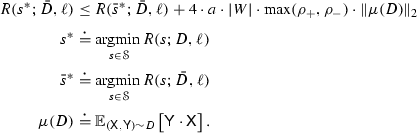Thus, as per Ghosh et al. (2015), their Theorem 10 bounds the corrupted risk, rather than clean regret, and does not establish consistency. Indeed, as the bound is in terms of the corrupted rather than clean distribution, it does not specify how well a solution obtained from the noisy distribution will perform on a test set comprising clean labels.

#### Comparison to Awasthi et al. (2015, 2017)

Awasthi et al. (2015, 2017) show that for separable $$D$$ with marginals possessing certain structure, one can guarantee small corrupted 0–1 regret for a specific algorithm under separable $$D$$. By contrast, the present work relates the clean and corrupted regret for the output of any algorithm, under no assumptions on the marginal distribution of $$D$$. Finally, these works provide no analysis of ranking consistency.

These works also provided an algorithm to provably learn under the settings of their theorems; however, to our knowledge, there has been no practical assessment of the performance of these methods. On the other hand, Awasthi et al. (2017) also provide analysis for settings beyond our label flipping noise model. It is an interesting topic for future work as to whether one can extend our analysis to such models.

### Comparison to regression approaches

Our LIN noise model is the natural discrete variant of heteroscedastic noise in regression problems (Le et al. 2005). Typically, such noise is handled by inferring the reliability of each instance, and then suitably weighting them (Shalizi 2017, Chapter 7). A distinct line of work has focussed on arbitrary (i.e., not necessarily probabilistically generated) regression noise (Wright and Ma 2010; Nguyen and Tran 2013; Bhatia et al. 2015). This is less immediately related to our probabilistic label-flipping noise setting.

## Experimental illustration of theoretical results

We present experiments that validate our theoretical results. While our primary contributions are in providing formal theoretical statements of the behaviour of learning algorithms under noise, we wish to illustrate that there are potential practical implications from our findings.

### Illustration of classification and ranking consistency

We first validate Theorems 1 and 2: we show that given access only to samples subject to instance dependent noise, a rich model can asymptotically-classify optimally; and if the noise is further boundary consistent, then it can rank optimally as well.

We fix a non-separable discrete distribution $$D$$ concentrated on notional instances $$\mathscr {X}= \{ x_1, x_2, \ldots , x_{16} \}$$. We assume a uniform marginal M, and set $$\eta ( x_i ) = i/16$$. We pick label flip function $$\rho ( x_i ) = \rho _{\mathrm {max}}$$ for $$i = 8$$ and $$\rho _{\mathrm {avg}}$$ otherwise, for parameters $$\rho _{\mathrm {max}}, \rho _{\mathrm {avg}}$$ to be specified. We then draw $$\bar{\mathsf {S}} \sim \bar{D}^m$$ from the induced corrupted distribution, compute the minimiser of the empirical logistic risk (since $$\mathscr {X}$$ is discrete, we can explicitly optimise over $$s \in \mathbb {R}^{16}$$), and compute the clean 0–1 regret of this solution. We repeat this for 100 random draws of of $$\bar{\mathsf {S}}$$.

We fix $$\rho _{\mathrm {max}} = 0.49$$, and vary $$\rho _{\mathrm {avg}} \in \{ 0.1, 0.2, 0.3, 0.4 \}$$. Figure 1 plots the average 0–1 regret as the number of samples m is varied. As predicted by Theorem 1, all the regrets eventually tend to zero; thus, asymptotically, we can classify optimally despite only having access to noisy samples. Further, as predicted by Eq. 7, small values of $$\rho _{\mathrm {avg}}$$ lead to significantly smaller 0–1 regret. This is despite the fact that all the induced noisy distributions $$\bar{D}$$ have the same maximal noise rate. Note now that $$\rho$$ is boundary consistent, since the noise is highest when $$\eta ( x ) = \nicefrac []{1}{2}$$. Figure 1 plots the average AUROC regret versus m, and confirms that this also tends to zero, as predicted by Theorem 2.

### Illustration of noise robustness of the Isotron

We next illustrate Theorem 3, showing that the Isotron can effectively learn GLMs under suitable boundary consistent (SIN) noise.

To start, we fix a non-separable $$D$$ such that M is a mixture of Gaussians with means (1, 1) and $$(-1, -1)$$ and identity covariance. We picked $$\eta ( x ) = \sigma ( s^*( x ) )$$ for sigmoid $$\sigma$$ and $$s^*( x ) = 10 \cdot x_1 + 10 \cdot x_2$$. For flip functions $$f_{\pm 1}( z ) = (1/2) e^{-z^2/4}$$, we drew a sample $$\bar{\mathsf {S}}$$ of 5000 elements from the boundary-consistent corruption of $$D$$. We then estimated $$\bar{\eta }$$ from $$\bar{\mathsf {S}}$$ using 1000 iterations of Isotron. Figure 1 shows this estimate closely matches the actual $$\bar{\eta }$$ computed explicitly via Eq. 4.

Next, we ran experiments on the USPS and MNIST datasets, for the tasks of distinguishing digits 0 and 9 for the former, and 6 and 7 for the latter. For an 80–20 train-test split, we inject boundary-consistent noise by flipping the training labels with probability $$f( x ) = \alpha \cdot \sigma ( \langle w^*, x \rangle ^2 )$$ for parameter $$\alpha \in [0, \nicefrac []{1}{2})$$, where $$w^*$$ is the optimal separator found by ordinary least squares. This mimics a scenario where the labels are from a human annotator liable to make errors for the easily confusable digits. We then trained regularised least squares and logistic regression models (using regularisation strength $$\lambda = 10^{-8}$$), and the Isotron (using 100 iterations) on the corrupted training sample. We measured the models’ classification accuracy on the test set with clean labels.

For $$\alpha \in \{ 0.0, 0.1, \ldots , 0.5 \}$$, Table 3 reports the mean and standard error of the accuracies over $$T = 25$$ independent corruptions for both datasets. We find that for higher $$\alpha$$ (i.e., more noise), the Isotron offers a significant improvement over standard learners.

### Further experiments with the Isotron

We now present results showing that the Isotron learns good decision boundaries on non-separable real-world datasets, and that it can estimate noise rates in class-conditional settings. This indicates that our results are not purely theoretical, and have potential practical viability; it also motivates further study of algorithms to learn SIMs, as they may lead to principled means of coping with instance-dependent noise.

#### UCI experiments

We first show that the boundary consistent noise (BCN) model captures the real-world labeling process to some extent, in that the Isotron can classify such data well. To this end, we run Isotron algorithm on several UCI benchmark datasets (preprocessed and made available by Gunnar RätschFootnote 2), using the given labels as is, without injecting any artificial noise. We compare the Isotron to two linear baseline methods, viz. ridge and logistic regression.

The results are presented in Table 4. We observe that in almost all the datasets, assuming a boundary consistent noise and using the Isotron helps learn a better linear decision boundary. This is so even when a linear model does not capture the underlying Bayes-optimal scorer, such as the highly non-linear banana dataset. Overall, this confirms the usefulness and conformance of the noise model.

#### Noise rate estimation

We additionally assessed the feasibility of using the Isotron to estimate noise rates for a class-conditional noise model, a possibility hinted at in Sect. 5.4. For the USPS and MNSIT datasets as used above, we artificially injected class-conditional noise with rate $$\rho _+ = 0.2$$ on instances from the positive class, and $$\rho _- = 0.4$$ from the negative class. We then used the quantile-based noise rate estimator of (Menon et al. 2015, Section 6.3) on the estimates of the corrupted probability $$\bar{\eta }$$ produced by the Isotron. Violin plots in Fig. 2 shows that on both datasets, the estimates of the noise rates are unbiased on average, with modest variance.

## Conclusion and future work

We have theoretically analysed the problem of learning with instance-dependent label noise, with three main conclusions:

1. (a)

for purely instance-dependent noise, minimising the classification risk on the noisy distribution is consistent for classification on the clean distribution;

2. (b)

for a broad class of “boundary consistent” label- and instance-dependent noise, a similar consistency result holds for the area under the ROC curve; and

3. (c)

one can learn generalised linear models subject to the same “boundary consistent” noise using the Isotron algorithm (Kalai and Sastry 2009).

For future work, determining sufficient conditions for order preservation of $$\eta$$, and studying simplified versions of the Isotron under more specific noise models (e.g., class-conditional) would be of interest.

1. 1.

It is crucial to use $$w^*$$ here, rather than any arbitrary w. With the latter, there would be no necessary connection between the level of noise and the underlying class-probability. As a result, the corrupted class-probabilities would not by themselves provide information about their clean counterparts.

2. 2.
3. 3.

This result is implicit in the proof of Ghosh et al. (2015, Theorem 1).

4. 4.

By contrapositive of Condition (a) of $$\mathrm {BCN}$$-admissibility, if $$s( x ) \le s( x' )$$ then $$\eta ( x ) \le \eta ( x' )$$.

5. 5.

If $$\eta \in \mathrm {SIM}( L, W )$$, then trivially $$\eta \in \mathrm {SIM}( 1, L \cdot W )$$, because $$\eta ( x ) = u( \langle w^*, x \rangle ) = u( (1/L) \cdot \langle (L \cdot w^*), x \rangle ) = \tilde{u}( \langle \tilde{w}^*, x \rangle )$$, where $$\tilde{u}$$ is a 1-Lipschitz function, and $$\Vert \tilde{w}^* \Vert _2 = L \cdot W$$.

## References

1. Agarwal, S. (2014). Surrogate regret bounds for bipartite ranking via strongly proper losses. Journal of Machine Learning Research, 15, 1653–1674.

2. Agarwal, S., & Niyogi, P. (2005). Stability and generalization of bipartite ranking algorithms. In Conference on learning theory (COLT), Springer (pp. 32–47).

3. Angluin, D., & Laird, P. (1988). Learning from noisy examples. Machine Learning, 2(4), 343–370.

4. Awasthi, P., Balcan, M. F., & Long, P. M. (2014). The power of localization for efficiently learning linear separators with noise. In Symposium on the theory of computing (STOC) (pp. 449–458).

5. Awasthi, P., Balcan, M. F., Haghtalab, N., & Urner, R. (2015). Efficient learning of linear separators under bounded noise. Conference on Learning Theory (COLT), 40, 167–190.

6. Awasthi, P., Balcan, M., Haghtalab, N., & Zhang, H. (2016). Learning and 1-bit compressed sensing under asymmetric noise. In Conference on learning theory (COLT) (pp. 152–192).

7. Awasthi, P., Balcan, M., & Long, P. M. (2017). The power of localization for efficiently learning linear separators with noise. Journal of the ACM, 63(6), 50.

8. Ayer, M., Brunk, H. D., Ewing, G. M., Reid, W. T., & Silverman, E. (1955). An empirical distribution function for sampling with incomplete information. The Annals of Mathematical Statistics, 26(4), 641–647.

9. Bartlett, P. L., Jordan, M. I., & McAuliffe, J. D. (2006). Convexity, classification, and risk bounds. Journal of the American Statistical Association, 101(473), 138–156.

10. Bhatia, K., Jain, P., & Kar, P. (2015). Robust regression via hard thresholding. In Advances in neural information processing systems (NIPS) (pp. 721–729).

11. Blum, A., & Mitchell, T. (1998). Combining labeled and unlabeled data with co-training. In Conference on learning theory (COLT) (pp. 92–100).

12. Blum, A., Frieze, A., Kannan, R., & Vempala, S.(1996). A polynomial-time algorithm for learning noisy linear threshold functions. In Foundations of computer science (FOCS) (pp. 330–338).

13. Bootkrajang, J. (2016). A generalised label noise model for classification in the presence of annotation errors. Neurocomputing, 192, 61–71.

14. Bootkrajang, J., & Kabán, A. (2014). Learning kernel logistic regression in the presence of class label noise. Pattern Recognition, 47(11), 3641–3655.

15. Bylander, T. (1994). Learning linear threshold functions in the presence of classification noise. In Conference on learning theory (COLT) (pp. 340–347).

16. Bylander, T. (1997). Learning probabilistically consistent linear threshold functions. In Conference on learning theory (COLT) (pp. 62–71).

17. Bylander, T. (1998). Learning noisy linear threshold functions (unpublished manuscript). http://www.cs.utsa.edu/~bylander/pubs/learning-noisy-ltfs.ps.gz.

18. Clémençon, S., Lugosi, G., & Vayatis, N. (2008). Ranking and empirical minimization of U-statistics. The Annals of Statistics, 36(2), 844–874.

19. Decatur, S. E. (1997). PAC learning with constant-partition classification noise and applications to decision tree induction. In International conference on machine learning (ICML) (pp. 83–91).

20. Devroye, L., Györfi, L., & Lugosi, G. (1996). A probabilistic theory of pattern recognition. Berlin: Springer.

21. Du, J., & Cai, Z. (2015). Modelling class noise with symmetric and asymmetric distributions. In Conference on artificial intelligence (AAAI) (pp. 2589–2595).

22. Elkan, C., & Noto, K. (2008). Learning classifiers from only positive and unlabeled data. In International conference on knowledge discovery and data mining (KDD) (pp. 213–220).

23. Frénay, B., & Kabán, A. (2014). A comprehensive introduction to label noise. In European symposium on artificial neural networks (ESANN) (pp. 667—676).

24. Frénay, B., & Verleysen, M. (2014). Classification in the presence of label noise: A survey. IEEE Transactions on Neural Networks and Learning Systems, 25(5), 845–869.

25. Ghosh, A., Manwani, N., & Sastry, P. S. (2015). Making risk minimization tolerant to label noise. Neurocomputing, 160, 93–107.

26. He, K., Zhang, X., Ren, S., & Sun, J. (2016). Deep residual learning for image recognition. In IEEE conference on computer vision and pattern recognition (CVPR) (pp. 770–778).

27. Jain, S., White, M., & Radivojac, P. (2016). Estimating the class prior and posterior from noisy positives and unlabeled data. In Advances in neural information processing systems (NIPS) (pp. 2685–2693).

28. Kakade, S., Kanade, V., Shamir, O., & Kalai, A.(2011). Efficient learning of generalized linear and single index models with isotonic regression. In Advances in neural information processing systems (NIPS) (pp. 927–935).

29. Kalai, A., & Sastry, R. (2009). The Isotron algorithm: High-dimensional isotonic regression. In Conference on learning theory (COLT).

30. Kalai, A., Klivans, A., Mansour, Y., & Servedio, R. (2005). Agnostically learning halfspaces. In Foundations of computer systems (FOCS) (pp. 11–20).

31. Koyejo, O. O., Natarajan, N., Ravikumar, P. K., & Dhillon, I. S. (2014). Consistent binary classification with generalized performance metrics. In Advances in neural information processing systems (NIPS) (pp. 2744–2752).

32. Krizhevsky, A., Sutskever, I., & Hinton, G. E. (2012). Imagenet classification with deep convolutional neural networks. In Advances in neural information processing systems (NIPS) (pp. 1106–1114).

33. Le, Q. V., Smola, A. J., & Canu, S. (2005). Heteroscedastic gaussian process regression. In International conference on machine learning (ICML) (pp. 489–496).

34. Ling, C. X., & Li, C. (1998). Data mining for direct marketing: Problems and solutions. In Knowledge discovery and data mining (KDD) (pp. 73–79).

35. Liu, T., & Tao, D. (2015). Classification with noisy labels by importance reweighting. IEEE Transactions on Pattern Analysis and Machine Intelligence, 2001, 447–461.

36. Long, P., & Servedio, R. (2008). Random classification noise defeats all convex potential boosters. In International conference on machine learning (ICML) (pp. 608–615).

37. Manwani, N., & Sastry, P. S. (2013). Noise tolerance under risk minimization. IEEE Transactions on Cybernetics, 43(3), 1146–1151.

38. Massart, P., & Nédélec, E. (2006). Risk bounds for statistical learning. The Annals of Statistics, 34(5), 2326–2366.

39. Menon, A. K., van Rooyen, B., Ong, C. S., & Williamson, B. (2015). Learning from corrupted binary labels via class-probability estimation. In International conference on machine learning (ICML) (pp. 125–134).

40. Narasimhan, H., Vaish, R., & Agarwal, S. (2014). On the statistical consistency of plug-in classifiers for non-decomposable performance measures. In Advances in neural information processing systems (NIPS) (pp. 1493–1501).

41. Natarajan, N., Dhillon, I. S., Ravikumar, P. D., & Tewari, A. (2013). Learning with noisy labels. In Advances in neural information processing systems (NIPS) (pp. 1196–1204).

42. Nguyen, N. H., & Tran, T. D. (2013). Exact recoverability from dense corrupted observations via $$\ell _1$$-minimization. IEEE Transactions on Information Theory, 59(4), 2017–2035.

43. Patrini, G., Nielsen, F., Nock, R., & Carioni, M.(2016). Loss factorization, weakly supervised learning and label noise robustness. In International conference on machine learning (ICML) (pp. 708–717).

44. Patrini, G., Rozza, A., Menon, A., Nock, R., & Qu, L. (2017). Making deep neural networks robust to label noise: A loss correction approach. In Computer vision and pattern recognition (CVPR) (pp. 2233–2241).

45. Plessis, M. C., Niu, G., Sugiyama, M. (2015). Convex formulation for learning from positive and unlabeled data. In International conference on machine learning (ICML) (pp. 1386–1394).

46. Ralaivola, L., Denis, F., & Magnan, C. N.(2006). CN = CPCN. In International conference on machine learning (ICML) (pp. 721–728).

47. Reed, S. E., Lee, H., Anguelov, D., Szegedy, C., Erhan, D., & Rabinovich, A. (2014). Training deep neural networks on noisy labels with bootstrapping. CoRR abs/1412.6596.

48. Reid, M. D., & Williamson, R. C.(2009). Surrogate regret bounds for proper losses. In International conference on machine learning (ICML) (pp. 897–904).

49. van Rooyen, B., Menon, A. K., & Williamson, R. C. (2015). Learning with symmetric label noise: the importance of being unhinged. In Advances in neural information processing systems (NIPS) (pp. 10–18).

50. Schölkopf, B., & Smola, A. J. (2001). Learning with kernels. Cambridge: MIT Press.

51. Scott, C., Blanchard, G., & Handy, G. (2013). Classification with asymmetric label noise: Consistency and maximal denoising. In Conference on learning theory (COLT) (pp. 489–511).

52. Servedio, R. (1999). On PAC learning using winnow, perceptron, and a perceptron-like algorithm. In Conference on learning theory (COLT) (pp. 296–307).

53. Shalizi, C. R. (2017). Advanced data analysis from an elementary point of view (unpublished book draft). http://www.stat.cmu.edu/~cshalizi/ADAfaEPoV/ADAfaEPoV.pdf.

54. Steinwart, I., & Scovel, C. (2005). Fast rates for support vector machines. In Conference on learning theory (COLT) (pp. 279–294).

55. Stempfel, G., & Ralaivola, L. (2007). Learning kernel perceptrons on noisy data using random projections. In Algorithmic learning theory (ALT) (pp. 328–342).

56. Stempfel, G., & Ralaivola, L. (2009). Learning SVMs from sloppily labeled data. In International conference on artificial neural networks (ICANN) (pp. 884–893).

57. Wright, J., & Ma, Y. (2010). Dense error correction via $$\ell _1$$-minimization. IEEE Transactions on Information Theory, 56(7), 3540–3560.

58. Xiao, T., Xia, T., Yang, Y., Huang, C., & Wang, X.(2015). Learning from massive noisy labeled data for image classification. In IEEE conference on computer vision and pattern recognition (CVPR) (pp. 2691–2699).

59. Zhang, T. (2004). Statistical behavior and consistency of classification methods based on convex risk minimization. Annals of Statistics, 32(1), 56–85.

## Author information

Authors

### Corresponding author

Editors: Jesse Davis, Elisa Fromont, Derek Greene, and Bjorn Bringmaan.

## Appendices

### Proof of Lemma 1

By definition of how corrupted labels $$\bar{\mathsf {Y}}$$ are generated,

\begin{aligned} \bar{\eta }( x )&= \mathbb {P}( \bar{\mathsf {Y}} = 1 \mid \mathsf {X}= x ) \\&= \sum _{y \in \{ \pm 1 \}} \mathbb {P}( \bar{\mathsf {Y}} = 1 \mid \mathsf {Y}= y, \mathsf {X}= x ) \cdot \mathbb {P}( \mathsf {Y}= y \mid \mathsf {X}= x ) \\&= (1 - \rho _1( x ) ) \cdot \eta ( x ) + \rho _{-1}( x ) \cdot ( 1 - \eta (x) ). \end{aligned}

The second identity follows by rearranging. $$\square$$

### Proof of Corollary 1

This is a simple consequence of the fact that weighting a risk does not affect Bayes-optimal scorers. Formally, for any $$w :\mathscr {X}\rightarrow \mathbb {R}_+$$, let the weighted $$\ell$$-risk be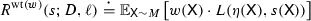(15)

where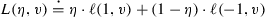. When $$w \equiv 1$$, this is the standard $$\ell$$-risk. By Proposition 4,

\begin{aligned} \underset{s}{{\text {argmin }}\, } R( s; D, \ell )&= \underset{s}{{\text {argmin }}\, } R^{\mathrm {wt}( w )}( s; \bar{D}, \ell ) \\&= \underset{s}{{\text {argmin }}\, } R( s; \bar{D}, \ell ). \end{aligned}

The second line is because weighting does not affect the Bayes-optimal scorers for a risk: for any $$w > 0$$,

\begin{aligned} ( \forall \eta \in [0, 1] ) \, \underset{v}{{\text {argmin }}\, } w \cdot L( \eta , v ) = \underset{v}{{\text {argmin }}\, } L( \eta , v ) \end{aligned}
\begin{aligned} R( s; D, \ell ) = \mathbb {E}_{\mathsf {X}\sim M}\left[ L( \eta (\mathsf {X}), s(\mathsf {X}) ) \right] . \end{aligned}

Note finally that by definition, the weighting factor $$w( x ) = (1 - 2 \cdot f( x ))^{-1} \ge 1$$, and so no term is suppressed after weighting. (If $$w( x ) = 0$$ for some $$x \in \mathscr {X}$$, then any prediction would be optimal for that instance; thus, we would get containment rather than equality of minimisers.)$$\square$$

### Proof of Theorem 1

Let $$s^{*} \in \underset{s}{{\text {argmin }}\, } R( s; D, \ell )$$. Let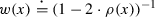, and recall $$R^{\mathrm {wt}( w )}$$ is the corresponding weighted $$\ell$$-risk (Eq. 15). By definition,

\begin{aligned} \mathrm {reg}\left( s; D, \ell \right)&= R( s; D, \ell ) - R( s^{*}; D, \ell ) \nonumber \\&= R^{\mathrm {wt}( w )}( s; \bar{D}, \ell ) - R^{\mathrm {wt}( w )}( s^{*}; \bar{D}, \ell ) \text { by Proposition } 4 \nonumber \\&= \mathbb {E}_{\mathsf {X}\sim M}\left[ \frac{1}{1 - 2 \cdot \rho ( \mathsf {X})} \cdot \left( L\left( \bar{\eta }( \mathsf {X}), s( \mathsf {X}) \right) - L\left( \bar{\eta }( \mathsf {X}), s^{*}( \mathsf {X}) \right) \right) \right] \text { by definition } \end{aligned}
(16)
\begin{aligned}&\le \frac{1}{1 - 2 \cdot \rho _{\mathrm {max}}} \cdot \mathbb {E}_{\mathsf {X}\sim M}\left[ L\left( \bar{\eta }( \mathsf {X}), s( \mathsf {X}) \right) - L\left( \bar{\eta }( \mathsf {X}), s^{*}( \mathsf {X}) \right) \right] \text { by Eq.}2 \nonumber \\&= \frac{1}{1 - 2 \cdot \rho _{\mathrm {max}}} \cdot \left( R\left( s; \bar{D}, \ell \right) - R\left( s^{*}; \bar{D}, \ell \right) \right) \nonumber \\&= \frac{1}{1 - 2 \cdot \rho _{\mathrm {max}}} \cdot \mathrm {reg}\left( s; \bar{D}, \ell \right) , \end{aligned}
(17)

where the last line is since by Corollary 1, we know that $$s^* \in \underset{s}{{\text {argmin }}\, } R( s; \bar{D}, \ell )$$ also. This fact also implies that for the inequality step above, we can guarantee $$L( \bar{\eta }( x ), s( x ) ) - L( \bar{\eta }( x ), s^{*}( x ) ) \ge 0$$ for every $$x \in \mathscr {X}$$, and so we do not have to worry about the direction of the inequality.

To get the second bound, suppose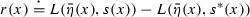is the conditional regret. Trivially,

\begin{aligned} r( x )&\le L( \bar{\eta }( x ), s( x ) ) \\&= \bar{\eta }( x ) \cdot \ell (1, s( x ) ) + (1 - \bar{\eta }( x )) \cdot \ell (1, s( x ) ) \\&\le B \text { by assumption on } \ell . \end{aligned}

Now define the (nonnegative) random variables $$\mathsf {W}= w( \mathsf {X})$$, $$\mathsf {R}= r( \mathsf {X})$$. The regret of Eq. 16 can be rewritten

\begin{aligned} \mathrm {reg}\left( s; D, \ell \right)&= \mathbb {E}_{\mathsf {X}\sim M}\left[ w( \mathsf {X}) \cdot r( \mathsf {X}) \right] \\&= \mathbb {E}_{}\left[ \mathsf {W}\cdot \mathsf {R} \right] \\&\le \left( \mathbb {E}_{}\left[ \mathsf {W}^{\frac{1}{\alpha }} \right] \right) ^\alpha \cdot \left( \mathbb {E}_{}\left[ \mathsf {R}^{\frac{1}{1 - \alpha }} \right] \right) ^{1 - \alpha } \text { by H}\ddot{\text {o}}\text {lder's inequality} \\&= W \cdot B \cdot \left( \mathbb {E}_{}\left[ \left( \frac{\mathsf {W}}{W} \right) ^{\frac{1}{\alpha }} \right] \right) ^\alpha \cdot \left( \mathbb {E}_{}\left[ \left( \frac{\mathsf {R}}{B} \right) ^{\frac{1}{1 - \alpha }} \right] \right) ^{1 - \alpha } \text { for } W = \max _x w( x ) \\&\le W \cdot B \cdot \left( \mathbb {E}_{}\left[ \frac{\mathsf {W}}{W} \right] \right) ^\alpha \cdot \left( \mathbb {E}_{}\left[ \frac{\mathsf {R}}{B} \right] \right) ^{1 - \alpha } \text { since } z^\beta \le z \text { for } z \in [ 0, 1 ], \beta \ge 1 \\&= W^{1-\alpha } \cdot B^{\alpha } \cdot \left( \mathbb {E}_{}\left[ \mathsf {W} \right] \right) ^\alpha \cdot \left( \mathbb {E}_{}\left[ \mathsf {R} \right] \right) ^{1 - \alpha } \\&= W^{1-\alpha } \cdot B^{\alpha } \cdot \left( \mathbb {E}_{\mathsf {X}\sim M}\left[ (1 - 2 \cdot \rho ( \mathsf {X}))^{-1} \right] \right) ^\alpha \cdot \left( \mathrm {reg}\left( s; \bar{D}, \ell \right) \right) ^{1 - \alpha }. \end{aligned}

Note that $$W = (1 - 2 \cdot \rho _{\mathrm {max}})^{-1}$$ by definition. The case $$\alpha = 0$$ gives the original bound of Eq. 2. $$\square$$

### Proof of Proposition 1

Pick some $$x, x'$$ such that $$\eta ( x ) < \eta ( x ' )$$. Certainly $$s( x ) < s( x' )$$ since s is order preserving for $$\eta$$ by BCN-admissibility Condition (a). Thus, by Lemma 5,

\begin{aligned} \bar{\eta }( x ) - \bar{\eta }( x' ) \le \max \left( 1 - \rho _{-1}( x ) - \rho _{1}( x ), 1 - \rho _{-1}( x' ) - \rho _{1}( x' ) \right) \cdot \left( \eta ( x ) - \eta ( x' ) \right) . \end{aligned}

By the total noise assumption (Assumption 2), $$1 - \rho _{-1}( x ) - \rho _{1}( x ) > 0$$ for every x, and so the $$\max ( \cdot )$$ term above is $$> 0$$. Since $$\eta ( x ) - \eta ( x' ) < 0$$ by assumption, we conclude that $$\bar{\eta }( x ) - \bar{\eta }( x' ) < 0$$. $$\square$$

### Proof of Theorem 2

From Clémençon et al. (2008) and Agarwal (2014, Theorem 11),

\begin{aligned} \mathrm {reg}_{\mathrm {rank}}( s; D) = \frac{1}{2 \cdot \pi \cdot (1 - \pi )} \cdot \mathbb {E}_{\mathsf {X}\sim M, \mathsf {X}' \sim M}\left[ | \eta ( \mathsf {X}) - \eta ( \mathsf {X}' ) | \cdot \mathbb {I}( \eta ( \mathsf {X}) - \eta ( \mathsf {X}' ), s( \mathsf {X}) - s( \mathsf {X}' ) ) \right] \end{aligned}

where

\begin{aligned} \mathbb {I}( \Delta \eta , \Delta s ) = \llbracket \Delta \eta \cdot \Delta s < 0 \rrbracket + \nicefrac []{1}{2} \cdot \llbracket \Delta s = 0 \rrbracket . \end{aligned}

By the order-preservation assumption,

\begin{aligned} \eta ( x ) \ne \eta ( x' ) \implies \mathrm {sign}( \eta ( x ) - \eta ( x' ) ) = \mathrm {sign}( \bar{\eta }( x ) - \bar{\eta }( x' ) ). \end{aligned}
(18)

Thus, in this case, $$\mathrm {sign}( \Delta \eta ) = \mathrm {sign}( \Delta \bar{\eta })$$, and so $$\mathbb {I}( \Delta \eta , \Delta s ) = \mathbb {I}( \Delta \bar{\eta }, \Delta s )$$. When $$\eta ( x ) = \eta ( x' )$$, however, there is no guarantee on the relative values of $$\bar{\eta }( x )$$ and $$\bar{\eta }( x' )$$. But if $$\Delta \eta = 0$$, then the first term in $$\mathbb {I}$$ above is necessarily zero, while that for $$\Delta \bar{\eta }$$ can only be $$\ge 0$$. Thus, when $$\eta ( x ) \ne \eta ( x' )$$ we have

\begin{aligned} \mathbb {I}( \Delta \eta , \Delta s ) \le \mathbb {I}( \Delta \bar{\eta }, \Delta s ), \end{aligned}

and so, further applying the assumption on the difference between $$\eta$$ values,

\begin{aligned} \mathrm {reg}_{\mathrm {rank}}( s; D)&\le \frac{1}{2 \cdot \pi \cdot (1 - \pi )} \\&\quad \cdot \mathbb {E}_{\mathsf {X}\sim M, \mathsf {X}' \sim M}\left[ | \eta ( \mathsf {X}) - \eta ( \mathsf {X}' ) | \cdot \mathbb {I}( \bar{\eta }( \mathsf {X}) - \bar{\eta }( \mathsf {X}' ), s( \mathsf {X}) - s( \mathsf {X}' ) ) \right] \\&\le \frac{1}{2 \cdot \pi \cdot (1 - \pi )} \cdot \\&\quad C \cdot \mathbb {E}_{\mathsf {X}\sim M, \mathsf {X}' \sim M}\left[ | \bar{\eta }( \mathsf {X}) - \bar{\eta }( \mathsf {X}' ) | \cdot \mathbb {I}( \bar{\eta }( \mathsf {X}) - \bar{\eta }( \mathsf {X}' ), s( \mathsf {X}) - s( \mathsf {X}' ) ) \right] \\&= \frac{\bar{\pi }\cdot (1 - \bar{\pi })}{\pi \cdot (1 - \pi )} \cdot C \cdot \mathrm {reg}_{\mathrm {rank}}( s; \bar{D}). \end{aligned}

In the special case of the $$\mathrm {BCN}$$ model, order-preservation holds by Proposition 1. What remains then is the $$| \eta ( x ) - \eta ( x' ) |$$ term. Now, by Lemma 5,

\begin{aligned}&( \forall x, x' \in \mathscr {X}) \, \eta ( x ) \le \eta ( x' ) \implies \bar{\eta }( x ) - \bar{\eta }( x' ) \\&\quad \le \max \left( 1 - \rho _{-1}( x ) - \rho _{1}( x ), 1 - \rho _{-1}( x' ) - \rho _{1}( x' ) \right) \cdot \left( \eta ( x ) - \eta ( x' ) \right) . \end{aligned}

Consequently, when $$\eta ( x ) < \eta ( x' )$$, we have

\begin{aligned} \frac{\bar{\eta }( x ) - \bar{\eta }( x' )}{\eta ( x ) - \eta ( x' )}&\ge \max \left( 1 - \rho _{-1}( x ) - \rho _{1}( x ), 1 - \rho _{-1}( x' ) - \rho _{1}( x' ) \right) \\&\ge 1 - 2 \cdot \rho _{\mathrm {max}} \text { by assumption }. \end{aligned}

By swapping x and $$x'$$, an identical result holds if $$\eta ( x ) > \eta ( x' )$$. If $$\eta ( x ) = \eta ( x' )$$, we trivially have $$0 = | \eta ( x ) - \eta ( x' ) | \le | \bar{\eta }( x ) - \bar{\eta }( x' ) | \cdot (1 - 2 \cdot \rho _{\mathrm {max}})^{-1}$$. Thus, the regret bound holds with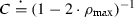. $$\square$$

### Proof of Proposition 2

By Lemma 2, the mandatory Condition (a) of the model $$\mathrm {BCN}( D, f_{-1}, f_{1}, s )$$ implies that $$\eta = u \circ s$$ for some non-decreasing u. Thus, by Lemma 1,

\begin{aligned} \bar{\eta }( x )&= \left( 1 - \rho _1( z ) \right) \cdot \eta ( x ) + \rho _{-1}( x ) \cdot \left( 1 - \eta ( x ) \right) \\&= \bar{u}\left( s( x ) \right) \end{aligned}

where

\begin{aligned} \bar{u}( z ) = \left( 1 - f_1( z ) \right) \cdot u( z ) + f_{-1}( z ) \cdot ( 1 - u ( z ) ). \end{aligned}

By Corollary 3,

\begin{aligned} s^*( x ) < s^*( x' ) \implies \bar{u}( s^*( x ) ) \le \bar{u}( s^*( x' ) ), \end{aligned}

so that $$\bar{u}$$ is a non-decreasing function, and thus a valid GLM link.

Next, applying the triangle inequality to Lemma 4, and using $$z = s( x ), z' = s( x' )$$,

\begin{aligned} | \bar{\eta }( x ) - \bar{\eta }( x' ) |&= | \bar{u}( z ) - \bar{u}( z' ) | \\&\le | 1 - f_{-1}( z' ) - f_{1}( z' ) | \cdot | u( z ) - u( z' ) | \\&\quad +|f_{-1}( z ) - f_{-1}( z' )| \cdot |1 - u(z)| + |f_{1}( z ) - f_{1}( z' )| \cdot |u(z)| \\&\le ( L + L_{-1} + L_{1} ) \cdot | z - z' |, \end{aligned}

using the fact that $$| 1 - f_{-1}( z' ) - f_{1}( z' ) | < 1$$ by the total noise assumption (Assumption 2), $$|1 - u(z)| \le 1$$ and $$|u(z)| \le 1$$ since $$\mathrm {Im}( u ) = [ 0, 1 ]$$, and the Lipschitz assumptions on $$u, f_{\pm 1}$$. It follows that $$\bar{u}$$ is $$( L + L_{-1} + L_{1} )$$-Lipschitz. $$\square$$

### Proof of Theorem 3

By Proposition 2, $$\bar{\eta }\in \mathrm {SIM}( L + L_2 + L_3, W )$$. Thus, as a member of the SIM family, it is suitable for estimation using SLIsotron.

Proposition 6 implies that one can always choose an iteration of SLIsotron with low regret. Let $$\hat{\bar{\eta }}_{\mathsf {S}, t}$$ denote the estimate produced by SLIsotron at iteration t. If in an abuse of notation we let $$\hat{\bar{\eta }}_{\mathsf {S}}$$ denote the estimate $$\hat{\bar{\eta }}_{\mathsf {S}, t^*}$$, where $$t^*$$ is an appropriately determined iteration, then we have that $$\mathrm {reg}( \hat{\bar{\eta }}_{\mathsf {S}}; D, \ell ^{\mathrm {sq}}) {\mathop {\rightarrow }\limits ^{\mathbb {P}}} 0$$.

For AUROC consistency, standard surrogate regret bounds (Agarwal 2014) imply that for any estimator $$\hat{\bar{\eta }}$$,

\begin{aligned} \mathrm {reg}_{\mathrm {rank}}\left( \hat{\bar{\eta }}; \bar{D}\right) \le \frac{1}{2 \cdot \bar{\pi }\cdot (1 - \bar{\pi })} \cdot \sqrt{\mathrm {reg}\left( \hat{\bar{\eta }}; \bar{D}, \ell ^{\mathrm {sq}}\right) } \end{aligned}

for $$\ell ^{\mathrm {sq}}$$ being the squared loss $$\ell ^{\mathrm {sq}}(y,v) = (1-yv)^2$$. By Theorem 2, we conclude that

\begin{aligned} \mathrm {reg}_{\mathrm {rank}}\left( \hat{\bar{\eta }}_{\bar{\mathsf {S}}}; D\right)&\le \frac{\bar{\pi }\cdot (1 - \bar{\pi })}{\pi \cdot (1 - \pi )} \cdot \frac{1}{1 - 2 \cdot \rho _{\mathrm {max}}} \cdot \mathrm {reg}_{\mathrm {rank}}\left( \hat{\bar{\eta }}_{\bar{\mathsf {S}}}; \bar{D}\right) \\&\le \frac{1}{2 \cdot \pi \cdot (1 - \pi )} \cdot \frac{1}{1 - 2 \cdot \rho _{\mathrm {max}}} \cdot \sqrt{\mathrm {reg}\left( \hat{\bar{\eta }}_{\bar{\mathsf {S}}}; \bar{D}, \ell ^{\mathrm {sq}}\right) }. \end{aligned}

The Isotron guarantee implies the RHS tends to 0 with sufficiently many samples. Thus, $$\mathrm {reg}_{\mathrm {rank}}( \hat{\bar{\eta }}_{\bar{\mathsf {S}}}; D) \rightarrow 0$$.

For classification consistency, standard surrogate regret bounds (Zhang 2004; Bartlett et al. 2006; Reid and Williamson 2009) imply that we can bound the 0–1 regret in terms of the square loss regret:

\begin{aligned} \mathrm {reg}\left( 2 \hat{\bar{\eta }} - 1; \bar{D}, \ell ^{01}\right) \le \sqrt{\mathrm {reg}\left( \hat{\bar{\eta }}; \bar{D}, \ell ^{\mathrm {sq}}\right) }. \end{aligned}

By Theorem 1, for symmetric (label-independent) noise, thresholding our estimate of $$\bar{\eta }$$ around $$\nicefrac []{1}{2}$$ yields:

\begin{aligned} \mathrm {reg}\left( c_{\bar{\mathsf {S}}}; D, \ell ^{01}\right)&= \mathrm {reg}\left( 2 \hat{\eta }_{\bar{\mathsf {S}}} - 1; D, \ell ^{01}\right) \\&\le \frac{1}{1 - 2 \cdot \rho _{\mathrm {max}}} \cdot \mathrm {reg}\left( 2 \hat{\eta }_{\bar{\mathsf {S}}} - 1; \bar{D}, \ell ^{01}\right) \\&= \frac{1}{1 - 2 \cdot \rho _{\mathrm {max}}} \cdot \sqrt{\mathrm {reg}\left( \hat{\bar{\eta }}_{\mathsf {S}}; \bar{D}, \ell ^{\mathrm {sq}}\right) }. \end{aligned}

The Isotron guarantee implies the RHS tends to 0 with sufficiently many samples. Thus, in the case of symmetric $$\mathrm {BCN}$$ noise, thresholding $$\bar{\eta }$$ around $$\nicefrac []{1}{2}$$ will be consistent wrt the clean distribution. $$\square$$

### B.1: Order preservation

We will make use of the following simple fact about order preservation, stated without proof.

### Lemma 2

Suppose $$f, g :\mathbb {R}\rightarrow \mathbb {R}$$ are such that

\begin{aligned} ( \forall x, y \in \mathbb {R}) \, f( x )< f( y ) \implies g( x ) < g( y ). \end{aligned}

Then, $$f = u \circ g$$ for some non-decreasing u.

Taking the contrapositive gives us an alternate useful statement.

### Corollary 2

Suppose $$f, g :\mathbb {R}\rightarrow \mathbb {R}$$ are such that

\begin{aligned} ( \forall x, y \in \mathbb {R}) \, g( x ) \le g( y ) \implies f( x ) \le f( y ). \end{aligned}

Then, $$f = u \circ g$$ for some non-decreasing u.

Finally, we can make a more precise statement about behaviour when $$g( x ) = g( y )$$ under the above conditions.

### Lemma 3

Suppose $$f, g :\mathbb {R}\rightarrow \mathbb {R}$$ are such that

\begin{aligned} ( \forall x, y \in \mathbb {R}) \, f( x )< f( y ) \implies g( x ) < g( y ). \end{aligned}

Then,

\begin{aligned} ( \forall x, y \in \mathbb {R}) \, g( x ) = g( y ) \implies f( x ) = f( y ). \end{aligned}
\begin{aligned} ( \forall x, y \in \mathbb {R}) \, g( x ) < g( y ) \implies f( x ) \le f( y ). \end{aligned}

### Proof

By the contrapositive in Corollary 2,

\begin{aligned} ( \forall x, y \in \mathbb {R}) \, g( x ) \le g( y ) \implies f( x ) \le f( y ). \end{aligned}

If $$g( x ) < g( y )$$ then trivially $$g( x ) \le g( y )$$ and the result follows. Suppose that $$g( x ) = g( y )$$. Then $$g( x ) \le g( y )$$ and $$g( y ) \le g( x )$$. Thus $$f( x ) \le f( y )$$ and $$f( y ) \le f( x )$$, i.e., $$f( x ) = f( y )$$. The result is also evident from the fact that $$f = u \circ g$$ by Corollary 2. $$\square$$

Note that if we only know that $$g( x ) < g( y ) \implies f( x ) \le f( y )$$, we cannot conclude that $$f = u \circ g$$, nor that $$g = u \circ f$$; we must be able to conclude something about the behaviour of f when $$g( x ) = g( y )$$.

### B.2: Relating clean and corrupt risks

We have the following general relationship between the risk on the clean and corrupted distributions, which is a generalisation of Natarajan et al. (2013, Lemma 1). In the following, we use the shorthand $$\ell _y( s ) = \ell ( y, s )$$.

### Proposition 3

Pick any distribution $$D$$, and any loss $$\ell$$. Suppose that $$\bar{D}= \mathrm {LIN}( D, \rho _1, \rho _{-1} )$$ for admissible $$\rho _{\pm 1} :\mathscr {X}\rightarrow [0, 1]$$. Then, for any scorer $$s :\mathscr {X}\rightarrow \mathbb {R}$$,

\begin{aligned} R( s; D, \ell ) = \mathbb {E}_{\mathsf {X}\sim M}\left[ w(\mathsf {X}) \cdot \left( (\bar{\eta }( \mathsf {X}) - \rho _{-1}( \mathsf {X})) \cdot \ell _{1}( s( \mathsf {X}) ) + (1 - \bar{\eta }( \mathsf {X}) - \rho _{1}( \mathsf {X}) ) \cdot \ell _{-1}( s( \mathsf {X}) ) ) \right) \right] \end{aligned}

where $$w( x ) = (1 - \rho _1( x ) - \rho _{-1}( x ))^{-1}$$.

### Proof of Proposition 3

Re-expressing Lemma 1, for $$\mathrm {LIN}( D, \rho _1, \rho _{-1} )$$,

\begin{aligned} ( \forall x \in \mathscr {X}) \, \eta ( x ) = w( x ) \cdot ( \bar{\eta }( x ) - \rho _{-1}( x ) ) \end{aligned}

and

\begin{aligned} ( \forall x \in \mathscr {X}) \, 1 - \eta ( x ) = w( x ) \cdot ( 1 - \bar{\eta }( x ) - \rho _{1}( x ) ), \end{aligned}

where $$w( x ) = (1 - \rho _1( x ) - \rho _{-1}( x ))^{-1} > 0$$. Thus, the $$\ell$$-risk of an arbitrary scorer is

\begin{aligned} R( s; D, \ell ) =&\ \mathbb {E}_{(\mathsf {X}, \mathsf {Y}) \sim D}\left[ \ell ( \mathsf {Y}, s(\mathsf {X}) ) \right] \nonumber \\ =&\ \mathbb {E}_{\mathsf {X}\sim M}\left[ \eta ( \mathsf {X}) \cdot \ell _{1}( s( \mathsf {X}) ) + (1 - \eta ( \mathsf {X}) ) \cdot \ell _{-1}( s( \mathsf {X}) ) \right] \nonumber \\ =&\ \mathbb {E}_{\mathsf {X}\sim M}\left[ w(\mathsf {X}) \cdot \left( (\bar{\eta }( \mathsf {X}) - \rho _{-1}( \mathsf {X})) \cdot \ell _{1}( s( \mathsf {X}) ) + (1 - \bar{\eta }( \mathsf {X}) - \rho _{1}( \mathsf {X}) ) \cdot \ell _{-1}( s( \mathsf {X}) ) ) \right) \right] . \end{aligned}
(19)

$$\square$$

The instantiation of Proposition 3 for the case of PIN noise and losses satisfying Eq. 5 will be useful in proving Corollary 1: in this case, we can show the clean risk is an instance-weighted version of the corrupted risk. Recall that for $$w :\mathscr {X}\rightarrow \mathbb {R}_+$$, $$R^{\mathrm {wt}(w)}$$ is the weighted $$\ell$$-risk, per Eq. 15. Then, we have the following.Footnote 3

### Proposition 4

Pick any distribution $$D$$, and loss $$\ell$$ satisfying Eq. 5. Suppose that $$\bar{D}= \mathrm {PIN}( D, \rho )$$ for admissible $$\rho :\mathscr {X}\rightarrow [0, \nicefrac []{1}{2})$$. Then, for any scorer $$s :\mathscr {X}\rightarrow \mathbb {R}$$,

\begin{aligned} R( s; D, \ell ) = R^{\mathrm {wt}( w )}( s; \bar{D}, \ell ) + A( D, \rho ) \end{aligned}

where $$w( x ) = ( 1 - 2 \cdot \rho ( x ) )^{-1}$$, and $$A( D, \rho )$$ is some term independent of s.

### Proof

(Proof of Proposition 4) By Proposition 3, for $$\mathrm {LIN}( D, \rho _1, \rho _{-1} )$$,

\begin{aligned} R( s; D, \ell )&= \mathbb {E}_{\mathsf {X}\sim M}\left[ w(\mathsf {X}) \cdot \left( \left( \bar{\eta }( \mathsf {X}) - \rho _{-1}( \mathsf {X})\right) \cdot \ell _{1}\left( s( \mathsf {X}) \right) + \left( 1 - \bar{\eta }( \mathsf {X}) - \rho _{1}( \mathsf {X}) \right) \cdot \ell _{-1}\left( s( \mathsf {X}) \right) \right) \right] \\&= \mathbb {E}_{\mathsf {X}\sim M}\left[ w(\mathsf {X}) \cdot \left( \bar{\eta }( \mathsf {X}) \cdot \ell _1\left( s(\mathsf {X}) \right) + \left( 1 - \bar{\eta }( \mathsf {X}) \right) \cdot \ell _{-1}\left( s( \mathsf {X}) \right) \right) \right] \\&\quad - \mathbb {E}_{\mathsf {X}\sim M}\left[ w(\mathsf {X}) \cdot \left( \rho _{-1}( \mathsf {X}) \cdot \ell _{1}\left( s( \mathsf {X}) \right) + \rho _{1}( \mathsf {X}) \cdot \ell _{-1}\left( s( \mathsf {X}) \right) \right) \right] \\&= \mathbb {E}_{\mathsf {X}\sim M}\left[ w(\mathsf {X}) \cdot \left( L\left( \bar{\eta }( \mathsf {X}), s( \mathsf {X}) \right) - \rho _{-1}( \mathsf {X}) \cdot \ell _{1}\left( s( \mathsf {X}) \right) - \rho _{1}\left( \mathsf {X}\right) \cdot \ell _{-1}\left( s( \mathsf {X}) \right) \right) \right] . \end{aligned}

If $$\rho _1 \equiv \rho _{-1} \equiv \rho$$, $$w( x ) = (1 - 2 \cdot \rho ( x ))^{-1}$$ and

\begin{aligned} R( s; D, \ell )&= \mathbb {E}_{\mathsf {X}\sim M}\left[ \frac{1}{1 - 2 \cdot \rho ( \mathsf {X})} \cdot L\left( \bar{\eta }( \mathsf {X}), s( \mathsf {X}) \right) \right] \\&\quad - \mathbb {E}_{\mathsf {X}\sim M}\left[ \frac{\rho ( \mathsf {X})}{1 - 2 \cdot \rho ( \mathsf {X})} \cdot \left( \ell _{1}\left( s( \mathsf {X}) \right) + \ell _{-1}\left( s( \mathsf {X}) \right) \right) \right] . \end{aligned}

Thus, if per assumption the sum of the partial losses is a constant C,

\begin{aligned} R( s; D, \ell ) = R^{\mathrm {wt}( w )}( s; \bar{D}, \ell ) - C \cdot \mathbb {E}_{\mathsf {X}\sim M}\left[ \frac{\rho ( \mathsf {X})}{1 - 2 \cdot \rho ( \mathsf {X})} \right] . \end{aligned}

Noting that the second term above does not depend on the scorer s, the result follows. $$\square$$

For the symmetric label noise model, Proposition 4 reduces to Natarajan et al. (2013, Theorem 9).

### B.3: Relating clean and corrupt thresholds

For a general LIN model, we have the following relation between the thresholds of $$\bar{\eta }$$ values and the corresponding thresholds for $$\eta$$.

### Proposition 5

Pick any distribution $$D$$. Suppose that $$\bar{D}= \mathrm {LIN}( D, \rho _{-1}, \rho _{1} )$$ for admissible $$\rho _{\pm 1} :\mathscr {X}\rightarrow [0, 1].$$ Then, for any $$t \in [0, 1]$$,

\begin{aligned} ( \forall x \in \mathscr {X}) \, \eta ( x )> t \iff \bar{\eta }( x ) > ( 1 - \rho _{1}( x ) - \rho _{-1}( x ) ) \cdot t + \rho _{-1}( x ). \end{aligned}

### Proof

(Proof of Proposition 5) By Lemma 1,

\begin{aligned} \eta ( x ) = \frac{\bar{\eta }( x ) - \rho _{-1}( x )}{1 - \rho _{1}( x ) - \rho _{-1}( x )}. \end{aligned}

By Assumption 2, $$\rho _1( x ) + \rho _{-1}( x ) < 1$$ for every $$x \in \mathscr {X}$$, and so $$1 - \rho _1( x ) - \rho _{-1}( x ) > 0$$. We thus have

\begin{aligned} \eta ( x )> t&\iff \frac{\bar{\eta }( x ) - \rho _{-1}( x )}{1 - \rho _{1}( x ) - \rho _{-1}( x )}> t \\&\iff {\bar{\eta }( x ) - \rho _{-1}( x )}> {(1 - \rho _{1}( x ) - \rho _{-1}( x ) \cdot t} \text { since } 1 - \rho _1( x ) - \rho _{-1}( x )> 0 \\&\iff {\bar{\eta }( x )} > ( 1 - \rho _{1}( x ) - \rho _{-1}( x ) ) \cdot t + \rho _{-1}( x ). \end{aligned}

$$\square$$

### B.4: Difference in $$\bar{\eta }$$ values

For the general LIN model, we have the following relation between the difference in $$\bar{\eta }$$ values and the corresponding $$\eta$$ values, which will be useful in demonstrating order preservation of $$\bar{\eta }$$ and $$\eta$$.

### Lemma 4

Pick any distribution $$D$$. Suppose $$\bar{D}= \mathrm {LIN}( D, \rho _{-1}, \rho _{1} )$$. Then,

\begin{aligned} \left( \forall x, x' \in \mathscr {X}\right) \, \bar{\eta }( x ) - \bar{\eta }( x' )&= \left( 1 - \rho _{-1}( x' ) - \rho _{1}( x' ) \right) \cdot \left( \eta ( x ) - \eta ( x' ) \right) + \Delta _1( x, x' ) \\&= \left( 1 - \rho _{-1}( x ) - \rho _{1}( x ) \right) \cdot \left( \eta ( x ) - \eta ( x' ) \right) + \Delta _2( x, x' ), \end{aligned}

where

\begin{aligned} \Delta _1( x, x' )&= \left( \rho _{-1}( x ) - \rho _{-1}( x' )\right) \cdot \left( 1 - \eta ( x )\right) + \left( \rho _{1}( x' ) - \rho _{1}( x )\right) \cdot \eta ( x ) \\ \Delta _2( x, x' )&= \left( \rho _{-1}( x ) - \rho _{-1}( x' )\right) \cdot \left( 1 - \eta ( x' )\right) + \left( \rho _{1}( x' ) - \rho _{1}( x )\right) \cdot \eta ( x' ). \end{aligned}

### Proof of Lemma 4

By Lemma 1,

\begin{aligned} \bar{\eta }( x )&= \left( 1 - \rho _1( x ) - \rho _{-1}( x ) \right) \cdot \eta ( x ) + \rho _{-1}( x ). \end{aligned}

Thus,

\begin{aligned} \bar{\eta }( x ) - \bar{\eta }( x' )&= \left( 1 - \rho _{-1}( x ) - \rho _{1}( x ) \right) \cdot \eta ( x ) - \left( 1 - \rho _{-1}( x' ) - \rho _{1}( x' ) \right) \cdot \eta ( x' ) \nonumber \\&\quad + \rho _{-1}( x ) - \rho _{-1}( x' ) \nonumber \\&= \left( 1 - \rho _{-1}( x' ) - \rho _{1}( x' ) \right) \cdot \left( \eta ( x ) - \eta ( x' ) \right) + \Delta _1( x, x' ), \end{aligned}
(20)

where

\begin{aligned} \Delta _1( x, x' )&= \left( \rho _{-1}( x' ) + \rho _{1}( x' ) - \rho _{-1}( x ) - \rho _{1}( x )\right) \cdot \eta ( x ) + \left( \rho _{-1}( x ) - \rho _{-1}( x' )\right) \\&= \left( \rho _{-1}( x ) - \rho _{-1}( x' )\right) \cdot \left( 1 - \eta ( x )\right) + \left( \rho _{1}( x' ) - \rho _{1}( x )\right) \cdot \eta ( x ). \end{aligned}

Alternately, we have

\begin{aligned} \bar{\eta }( x ) - \bar{\eta }( x' )&= \left( 1 - \rho _{-1}( x ) - \rho _{1}( x ) \right) \cdot \left( \eta ( x ) - \eta ( x' ) \right) + \Delta _2( x, x' ) \end{aligned}
(21)

where

\begin{aligned} \Delta _2( x, x' )&= \left( \rho _{-1}( x' ) - \rho _{-1}( x ) + \rho _{1}( x' ) - \rho _{1}( x )\right) \cdot \eta ( x' ) + \left( \rho _{-1}( x ) - \rho _{-1}( x' )\right) \\&= \left( \rho _{-1}( x ) - \rho _{-1}( x' )\right) \cdot \left( 1 - \eta ( x' )\right) + \left( \rho _{1}( x' ) - \rho _{1}( x )\right) \cdot \eta ( x' ). \end{aligned}

$$\square$$

Some examples illustrate the above result.

### Example 6

For the case of class-conditional noise where $$\rho _1 \equiv \alpha , \rho _{-1} \equiv \beta$$, $$\Delta _1 \equiv \Delta _2 \equiv 0$$ and so we have the simpler expression

\begin{aligned} \bar{\eta }( x ) - \bar{\eta }( x' ) = \left( 1 - \alpha - \beta \right) \cdot \left( \eta ( x ) - \eta ( x' ) \right) , \end{aligned}

from which order preservation is immediate.

### Example 7

For the case of purely instance-dependent noise $$\mathrm {PIN}( D, \rho )$$,

\begin{aligned} \Delta _1( x, x' )&= \left( \rho ( x ) - \rho ( x' ) \right) \cdot \left( 1 - 2 \cdot \eta ( x ) \right) \\ \Delta _2( x, x' )&= \left( \rho ( x ) - \rho ( x' ) \right) \cdot \left( 1 - 2 \cdot \eta ( x' ) \right) . \end{aligned}

Thus,

\begin{aligned} \bar{\eta }( x ) - \bar{\eta }( x' )&= \left( 1 - 2 \cdot \rho ( x' )\right) \cdot \left( \eta ( x ) - \eta ( x' )\right) + \left( \rho ( x ) - \rho ( x' ) \right) \cdot \left( 1 - 2 \cdot \eta ( x ) \right) \\&= \left( 1 - 2 \cdot \rho ( x )\right) \cdot \left( \eta ( x ) - \eta ( x' )\right) + \left( \rho ( x ) - \rho ( x' ) \right) \cdot \left( 1 - 2 \cdot \eta ( x' ) \right) . \end{aligned}

Order preservation here will depend on the structure of $$\rho$$.

For the BCN model, Lemma 4 can be converted to show that $$\bar{\eta }$$ is a monotone transform of s, the underlying score used in the noise model; furthermore, we have a simple bound on the differences in $$\bar{\eta }$$ values in terms of the corresponding difference in $$\eta$$ values.

### Lemma 5

Pick any distribution $$D$$. Suppose $$\bar{D}= \mathrm {BCN}( D, f_{-1}, f_{1}, s )$$ where $$( f_{-1}, f_{1}, s, \eta )$$ are $$\mathrm {BCN}$$-admissible. Then,

\begin{aligned} \left( \forall x, x' \in \mathscr {X}\right) \, s( x )\le & {} s( x' ) \implies \bar{\eta }( x ) - \bar{\eta }( x' ) \\\le & {} \max \left( 1 - \rho _{-1}( x ) - \rho _{1}( x ), 1 - \rho _{-1}( x' ) - \rho _{1}( x' ) \right) \cdot \left( \eta ( x ) - \eta ( x' ) \right) \end{aligned}

where $$\rho _{\pm 1}( x ) = f_{\pm 1} \circ s$$. Further, if $$s( x ) = s( x' )$$, then $$\bar{\eta }( x ) = \bar{\eta }( x' )$$.

### Proof

(Proof of Lemma 5) Note that by Condition (a) of BCN-admissiblity and Lemma 2, $$\eta = u \circ s$$ for some non-decreasing u. For the BCN model, Lemma 4 is

\begin{aligned} ( \forall x, x' \in \mathscr {X}) \, \bar{\eta }( x ) - \bar{\eta }( x' )&= ( 1 - f_{-1}( z' ) - f_{1}( z' ) ) \cdot ( u( z ) - u( z' ) ) + \Delta _1( z, z' ) \\&= ( 1 - f_{-1}( z ) - f_{1}( z ) ) \cdot ( u( z ) - u( z' ) ) + \Delta _2( z, z' ), \end{aligned}

where $$z = s( x ), z' = s( x' )$$, and

\begin{aligned} \Delta _1( z, z' )&= (f_{-1}( z ) - f_{-1}( z' )) \cdot (1 - u( z )) + (f_{1}( z' ) - f_{1}( z )) \cdot u( z ) \\ \Delta _2( z, z' )&= (f_{-1}( z ) - f_{-1}( z' )) \cdot (1 - u( z' )) + (f_{1}( z' ) - f_{1}( z )) \cdot u( z' ). \end{aligned}

Suppose that $$s( x ) = s( x' )$$. Then clearly $$\rho _y( x ) = \rho _y( x' )$$, implying that $$\Delta _1 \equiv \Delta _2 \equiv 0$$, and also $$u( z ) = u( z' )$$ by Corollary 2, so $$\bar{\eta }( x ) = \bar{\eta }( x' )$$.

Suppose that $$s( x ) < s( x' )$$ so thatFootnote 4 $$\eta ( x ) \le \eta ( x' )$$; or equivalently, $$z < z'$$ so that $$u( z ) \le u( z' )$$. Our goal is to show that $$\min ( \Delta _1( z, z' ), \Delta _2( z, z' ) ) \le 0$$; this will imply the desired bound, since we can just use the tighter of the implied bounds on Eqs. 20 and 21. By Condition (c) of $$\mathrm {BCN}$$-admissibility, for any $$z < z'$$,

\begin{aligned} f_{1}( z ) - f_{-1}( z ) \ge f_{1}( z' ) - f_{-1}( z' ) \end{aligned}

or equivalently

\begin{aligned} f_{1}( z' ) - f_{1}( z ) \le f_{-1}( z' ) - f_{-1}( z ). \end{aligned}
(22)

Thus, since $$u( z ) \ge 0$$, we have

\begin{aligned} \Delta _1( z, z' )&\le (f_{-1}( z ) - f_{-1}( z' )) \cdot (1 - 2 \cdot u( z )), \end{aligned}
(23)

and similarly,

\begin{aligned} \Delta _2( z, z' )&\le (f_{-1}( z ) - f_{-1}( z' )) \cdot (1 - 2 \cdot u( z' )). \end{aligned}
(24)

We now argue why the minimum of these terms must be $$\le 0$$. Consider the following three cases:

1. (a)

Suppose $$f_{-1}( z ) = f_{-1}( z' )$$. Then trivially both terms are $$\le 0$$.

2. (b)

Suppose $$f_{-1}( z ) < f_{-1}( z' )$$. Then either $$u( z ) \le \frac{1}{2}$$ or $$u( z' ) \le \frac{1}{2}$$; if both u values are larger than $$\frac{1}{2}$$, then by BCN-admissibility Condition (b) it must be true that $$f_{-1}( z ) \ge f_{-1}( z' )$$, a contradiction. Thus either $$1 - 2 \cdot u( z ) \ge 0$$ or $$1 - 2 \cdot u( z' ) \ge 0$$, and so one of the terms must be $$\le 0$$.

3. (c)

Suppose $$f_{-1}( z ) > f_{-1}( z' )$$. Then either $$u( z ) \ge \frac{1}{2}$$ or $$u( z' ) \ge \frac{1}{2}$$; if both u values are smaller than $$\frac{1}{2}$$, then by BCN-admissibility Condition (b) it must be true that $$f_{-1}( z ) \le f_{-1}( z' )$$, a contradiction. Thus either $$1 - 2 \cdot u( z ) \le 0$$ or $$1 - 2 \cdot u( z' ) \le 0$$, and so one of the terms must be $$\le 0$$.

Thus, we conclude $$\min ( \Delta _1( z, z' ), \Delta _2( z, z' ) ) \le 0$$, and so either

\begin{aligned} {\bar{\eta }( x ) - \bar{\eta }( x' )} \le ( 1 - \rho _{-1}( x ) - \rho _{1}( x ) ) \cdot ( \eta ( x ) - \eta ( x' ) ) \end{aligned}

or

\begin{aligned} {\bar{\eta }( x ) - \bar{\eta }( x' )} \le ( 1 - \rho _{-1}( x ) - \rho _{1}( x' ) ) \cdot ( \eta ( x ) - \eta ( x' ) ) \end{aligned}

must be true; since $$\eta ( x ) - \eta ( x' ) \le 0$$ and $$\max ( 1 - \rho _{-1}( x ) - \rho _{1}( x ), 1 - \rho _{-1}( x ) - \rho _{1}( x' ) ) > 0$$, this implies

\begin{aligned} {\bar{\eta }( x ) - \bar{\eta }( x' )} \le \max ( 1 - \rho _{-1}( x ) - \rho _{1}( x ), 1 - \rho _{-1}( x ) - \rho _{1}( x' ) ) \cdot ( \eta ( x ) - \eta ( x' ) ). \end{aligned}

Since $$\eta ( x ) - \eta ( x' ) \le 0$$ and $$\max ( 1 - \rho _{-1}( x ) - \rho _{1}( x ), 1 - \rho _{-1}( x ) - \rho _{1}( x' ) ) > 0$$, we may bound the entire expression by 0, thus concluding that $$\bar{\eta }( x ) \le \bar{\eta }( x' )$$.$$\square$$

An immediate consequence of Lemma 5 is that $$\bar{\eta }$$ is order-preserving for the underlying scores.

### Corollary 3

Suppose $$\bar{D}= \mathrm {BCN}( D, f_{-1}, f_{1}, s )$$ where $$( f_{-1}, f_{1}, s, \eta )$$ are $$\mathrm {BCN}$$-admissible. Then,

\begin{aligned} ( \forall x, x' \in \mathscr {X}) \, s( x ) \le s( x' ) \implies \bar{\eta }( x ) \le \bar{\eta }( x' ) \end{aligned}

and so $$\bar{\eta }= \bar{u} \circ s$$ for some non-decreasing $$\bar{u}$$.

### Proof

By Lemma 5, if $$s( x ) = s( x' )$$ then $$\bar{\eta }( x ) = \bar{\eta }( x' )$$. If $$s( x ) < s( x' )$$ then $$\eta ( x ) \le \eta ( x' )$$ by BCN-admissiblity Condition (a). Further, $$1 - \rho _1( x ) - \rho _{-1}( x ) > 0$$ by Assumption 2. Thus, $$\bar{\eta }( x ) - \bar{\eta }( x' ) \le 0$$. The fact that $$\bar{\eta }= \bar{u} \circ s$$ follows from Corollary 2.$$\square$$

### Remark 1

By definition of BCN admissibility, $$\eta = u \circ s$$ for some monotone u; and by Lemma 5, $$\bar{\eta }= \bar{u} \circ s$$, for some monotone $$\bar{u}$$. If we could establish that $$\bar{u}$$ were strictly monotone, then we would immediately conclude $$\eta = u \circ \bar{u}^{-1} \circ \bar{\eta }$$, which would establish Proposition 1. But this is not true in general; fortunately, $$\bar{u}$$ is only constant when u is (owing to the explicit bound in Lemma 5), and so we are still able to write $$\eta = \phi \circ \bar{\eta }$$ for some monotone $$\phi$$.

### Remark 2

Order preservation by itself does not let us establish an AUROC regret bound. We need the precise bound on the difference in $$\bar{\eta }$$ values provided in Lemma 5 to quantify how much distortion is introduced relative to the difference in $$\eta$$ values.

### B.5: Class-probability estimation guarantees with the Isotron

We recall that the basic SLIsotron guarantee is as follows.

### Proposition 6

(Kakade et al. 2011, Theorem 2) Pick any distribution $$D$$ over $$\mathbb {B}^d \times \{ \pm 1 \}$$ withFootnote 5 $$\eta \in \mathrm {SIM}( 1, W )$$ for some $$W \in \mathbb {R}_+$$. Let $$\{ \hat{\eta }_{\mathsf {S}, t} \}_{t = 1}^\infty$$ denote the estimates of $$\eta$$ produced at each iteration of SLISotron, when applied to a training sample $$\mathsf {S} \sim D^m$$. Then, for any $$\delta \in ( 0, 1 )$$,

\begin{aligned} \mathbb {P}_{\mathsf {S} \sim D^m}\left( \min _{t} \mathrm {reg}\left( \hat{\eta }_{\mathsf {S}, t}; D, \ell ^{\mathrm {sq}}\right) \le \left( \frac{dW^2}{m} \right) ^{1/3} \cdot \left( \log \frac{Wm}{\delta } \right) ^{1/3} \right) \ge 1 - \delta \end{aligned}

where

\begin{aligned} \mathrm {reg}\left( \hat{\eta }; D, \ell ^{\mathrm {sq}}\right) = \mathbb {E}_{\mathsf {X}\sim M}\left[ ( \hat{\eta }( \mathsf {X}) - \eta ( \mathsf {X}) )^2 \right] . \end{aligned}

### C: Failure of order preservation under $$\bar{\eta }$$

We illustrate that for noise models other than $$\mathrm {BCN}$$, order preservation under $$\bar{\eta }$$ is not guaranteed.

### C.1: Failure of order preservation without Condition (c)

Order preservation is not guaranteed without Condition (c) of the $$\mathrm {BCN}$$ model.

### Example 8

Suppose $$f_{1}( z ) \equiv 0$$, $$f_{-1}( z ) = a \cdot \llbracket z \le 0 \rrbracket$$ for some $$a < 1$$, and s is such that $$\eta ( x ) = \frac{1}{1 + e^{-s( x )}}$$. Certainly $$( f_{-1}, f_{1}, s )$$ satisfy the requisite Conditions (a), (b) of the $$\mathrm {BCN}$$ model. However, $$f_1( z ) - f_{-1}( z )$$ is non-decreasing, and so Condition (c) is not satisfied. It is easy to check that

\begin{aligned} \bar{\eta }( x )&= \varphi ( s( x ) ) \\ \varphi ( z )&= \left( 1 - a \cdot \llbracket z \le 0 \rrbracket \right) \cdot \frac{e^z}{1 + e^z} + a \cdot \llbracket z \le 0 \rrbracket \\&= {\left\{ \begin{array}{ll} (1 - a) \cdot \frac{e^z}{1 + e^z} + a &{}\quad \text { if } z \le 0 \\ \frac{e^z}{1 + e^z} &{}\quad \text { if } z > 0, \end{array}\right. } \end{aligned}

which is easily checked to not be monotone in z.

The difference $$\Delta ( z ) = f_1( z ) - f_{-1}( z )$$ above is non-decreasing. Swapping the flip functions thus makes the function non-increasing, satisfying Condition (c) of the $$\mathrm {BCN}$$ model. We can confirm that in this case, $$\bar{\eta }$$ will indeed be order-preserving for $$\eta$$.

### Example 9

Suppose $$f_{-1}( z ) \equiv 0$$, $$f_{1}( z ) = a \cdot \llbracket z \le 0 \rrbracket$$ for some $$a < 1$$, and s is such that $$\eta ( x ) = \frac{1}{1 + e^{-s( x )}}$$. Certainly $$( f_{-1}, f_{1}, s )$$ satisfy the requisite Conditions (a), (b) of the $$\mathrm {BCN}$$ model. It is easy to check that

\begin{aligned} \bar{\eta }( x )&= \varphi ( s( x ) ) \\ \varphi ( z )&= \left( 1 - a \cdot \llbracket z \le 0 \rrbracket \right) \cdot \frac{e^z}{1 + e^z} \\&= {\left\{ \begin{array}{ll} a \cdot \frac{e^z}{1 + e^z} &{}\quad \text { if } z \le 0 \\ \frac{e^z}{1 + e^z} &{}\quad \text { if } z > 0, \end{array}\right. } \end{aligned}

which is easily checked to be monotone in z.

Condition (c) implies an asymmetry in the treatment of the positive and negative labels. When $$f_1 - f_{-1}$$ is non-decreasing rather than non-increasing, one may think to resolve this by simply swapping the roles of the positive and negative labels. Why is there an asymmetry, and why will this approach not work?

The reason is that the underlying score $$s^*$$ is such that $$\eta = u \circ s^*$$ for some non-decreasing $$u( \cdot )$$, so that higher scores correspond to equal or higher probability of an example being positive. This already imposes some restriction on how the scores relate to the labels, and so the flip functions must respect this.

In particular, suppose $$f_1 - f_{-1}$$ is non-decreasing, but we just relabel the positives as negatives and vice-versa. Certainly then our new $$\tilde{f}_1 - \tilde{f}_{-1}$$ on the relabelled positive and negative classes will be non-increasing. However, we also have new class-probability $$\tilde{\eta } = \tilde{u} \cdot s$$, where now the link is non-increasing. This means that s actually is reverse order preserving, and so we cannot conclude that the resulting $$\tilde{\bar{\eta }}$$ will be order preserving for $$\eta$$.

### C.2: Failure of order preservation for the PIN model

For the PIN model, order preservation will not be guaranteed in general. This means that it does not suffice to merely remove dependence of the noise on the labels.

### Example 10

Consider a model $$\mathrm {PIN}(D, \rho )$$ where $$\rho ( x ) = \frac{1}{2} \cdot \eta ( x )$$. This means that there is more noise for positive instances. Then, we have

\begin{aligned} \bar{\eta }( x )&= (1 - \eta ( x )) \cdot \eta ( x ) + \frac{1}{2} \cdot \eta ( x ) \\&= \eta ( x ) \cdot \left( \frac{3}{2} - \eta ( x ) \right) . \end{aligned}

This will not be order preserving for $$\eta$$, since $$\varphi ( z ) = z \cdot \left( \frac{3}{2} - z \right)$$ is not monotone on [0, 1].

Example 10 violates Condition (b), illustrating why this is important for guaranteeing order preservation.

## Rights and permissions

Reprints and Permissions